libxlsxwriter
Working with Charts

This section explains how to work with some of the options and features of The Chart object.

The majority of the examples in this section are based on a variation of the following program:

int main() {
lxw_workbook *workbook = workbook_new("chart_line.xlsx");
lxw_chart *chart;
/* Write some data for the chart. */
worksheet_write_number(worksheet, 0, 0, 10, NULL);
worksheet_write_number(worksheet, 1, 0, 40, NULL);
worksheet_write_number(worksheet, 2, 0, 50, NULL);
worksheet_write_number(worksheet, 3, 0, 20, NULL);
worksheet_write_number(worksheet, 4, 0, 10, NULL);
worksheet_write_number(worksheet, 5, 0, 50, NULL);
/* Create a chart object. */
/* Configure the chart. */
(void)series; /* Do something with series in the real examples. */
/* Insert the chart into the worksheet. */
worksheet_insert_chart(worksheet, CELL("C1"), chart);
return workbook_close(workbook);
}# Chart Value and Category Axes

When working with charts it is important to understand how Excel differentiates between a chart axis that is used for series categories and a chart axis that is used for series values.

In the majority of Excel charts the X axis is the category axis and each of the values is evenly spaced and sequential. The Y axis is the value axis and points are displayed according to their value: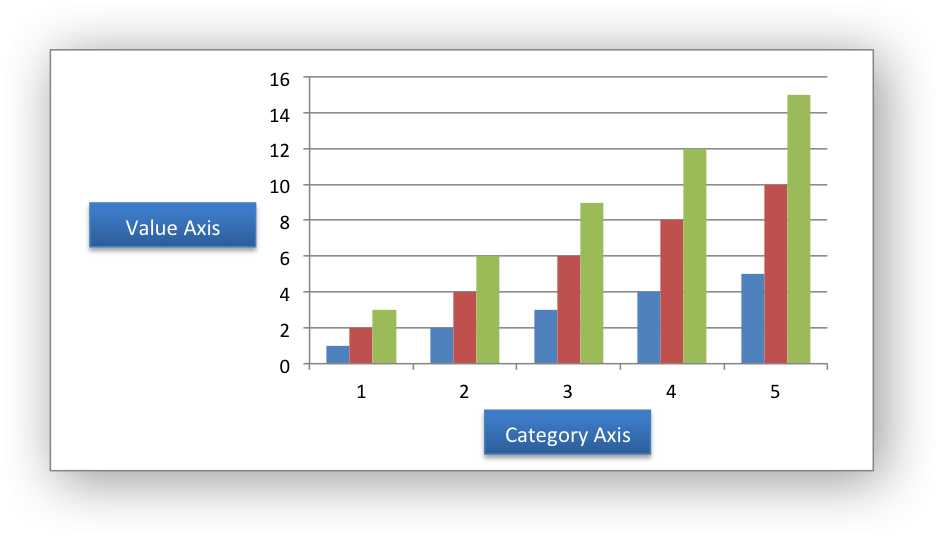Excel treats these two types of axis differently and exposes different properties for each. For example, here are the properties for a category axis: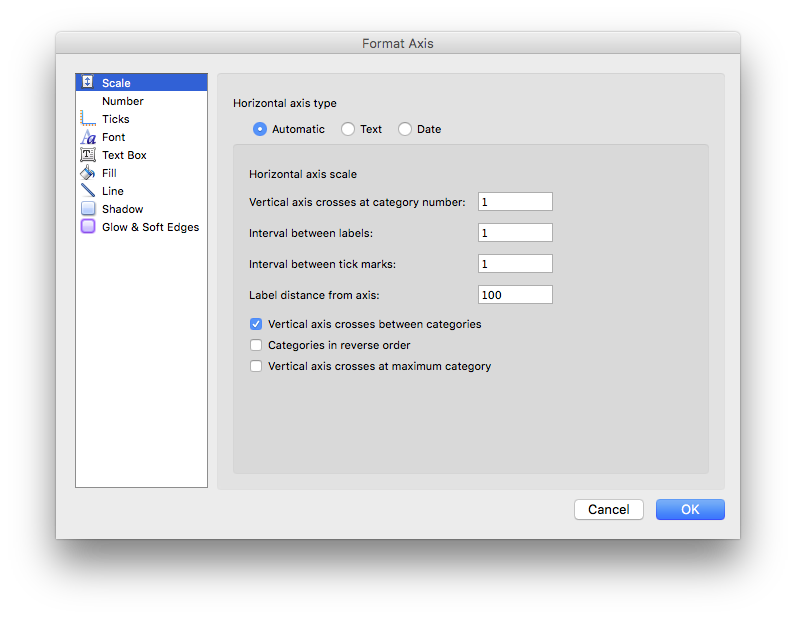Here are properties for a value axis: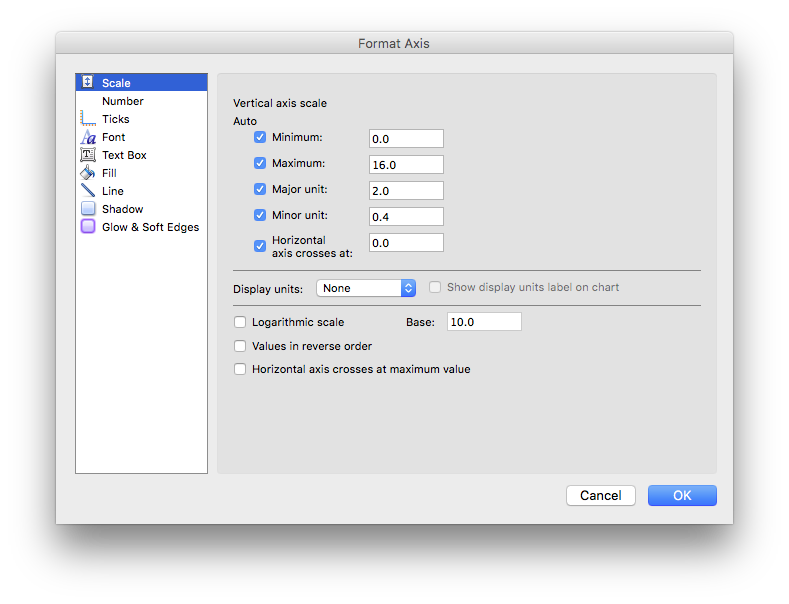As such, some of the `libxlsxwriter` axis properties can be set for a value axis, some can be set for a category axis and some properties can be set for both. The documentation calls out the type of axis to which functions apply and the API emits a warning if the wrong type is used.

Some category axes, such as the one in the column chart example above, use numbers as the categories. However, these numbers can't be treated like value axes. In particular you can't use chart_axis_set_max() and chart_axis_set_min() for category axes, as you can see in the respective format dialogs above.

For a Bar chart the Category and Value axes are reversed: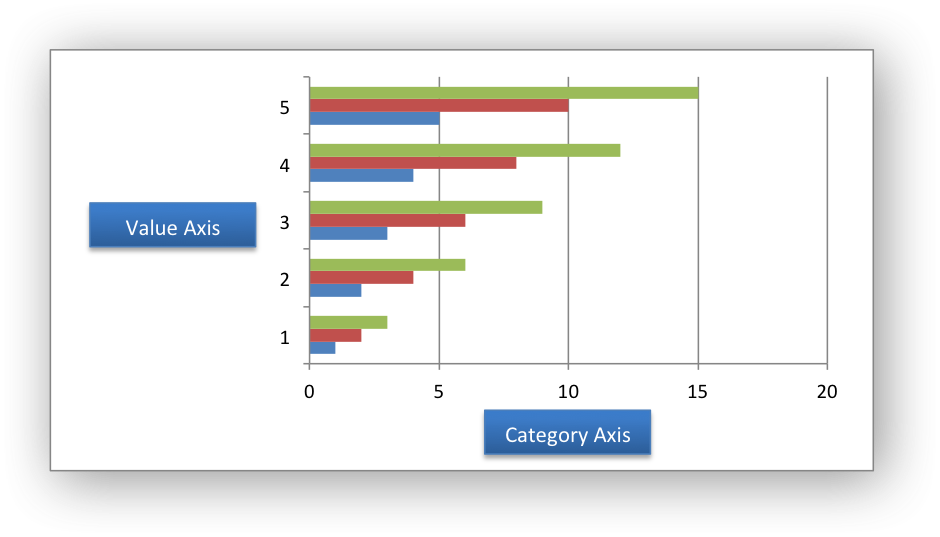A Scatter chart (but not a Line chart) has 2 value axes: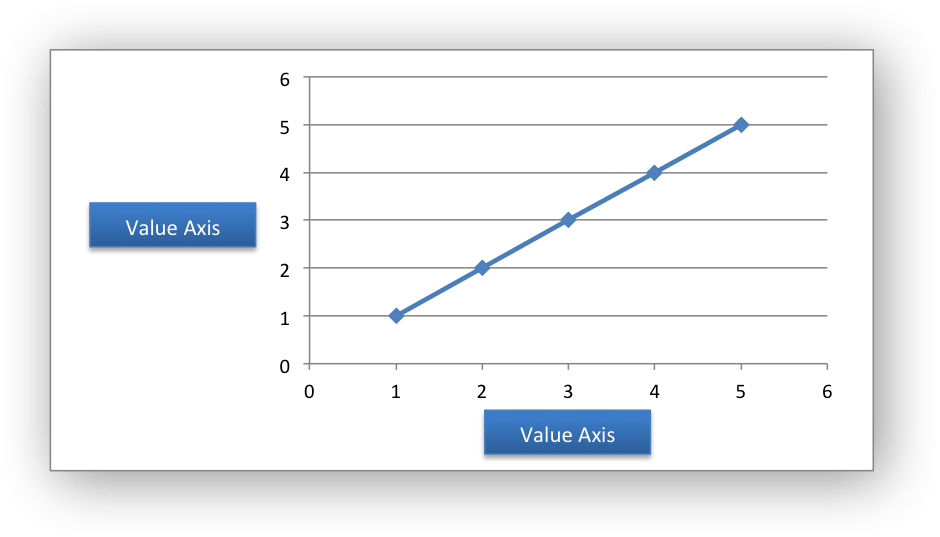Note
Date axes aren't supported yet.

# Working with Chart Markers

In Excel a chart marker is used to distinguish data points in a plotted series. In general only Line and Scatter and Radar chart types use markers. The libxlsxwriter chart types that can have markers are:

The chart types with `MARKERS` in the name have markers with default colors and shapes turned on by default but it is possible to change them using the functions shown below.

The `chart_series_set_marker_type()` function is used to specify the type of the series marker: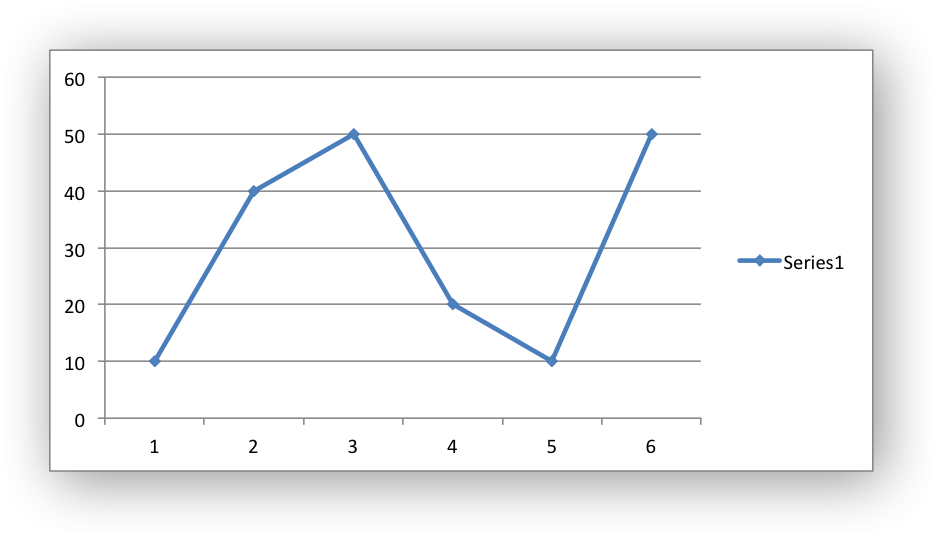The available marker types defined by lxw_chart_marker_type are:

The `#LXW_CHART_MARKER_NONE` type can be used to turn off default markers: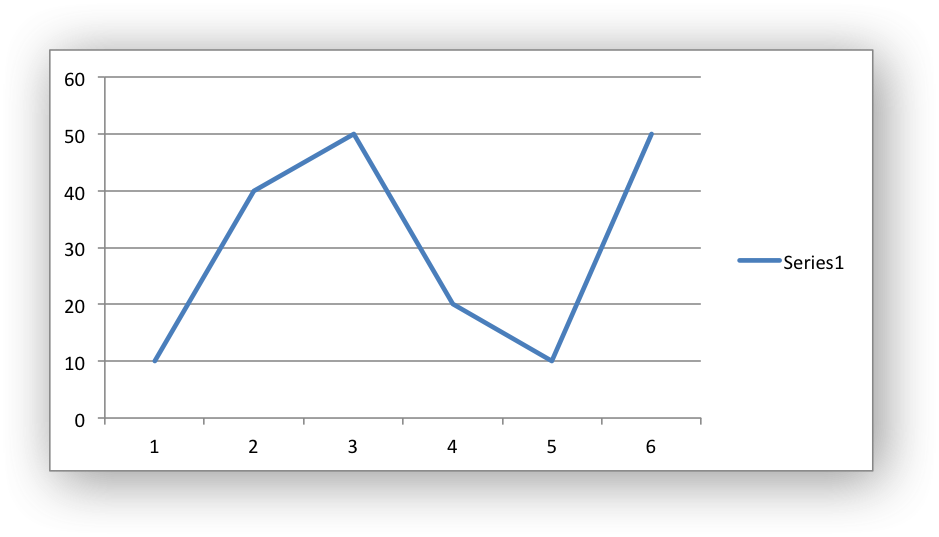The `#LXW_CHART_MARKER_AUTOMATIC` type is a special case which turns on a marker using the default marker style for the particular series. If automatic is on then other marker properties such as size, line or fill cannot be set.

The `chart_series_set_marker_size()` function is used to specify the size of the series marker: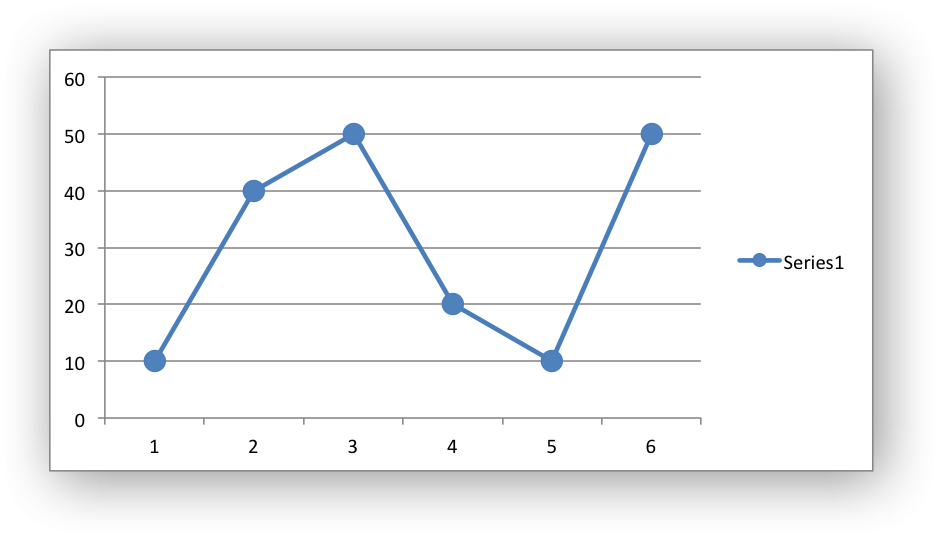The `chart_series_set_marker_line()` and `chart_series_set_marker_fill()` functions can be used to set the line/border and fill properties of a chart marker: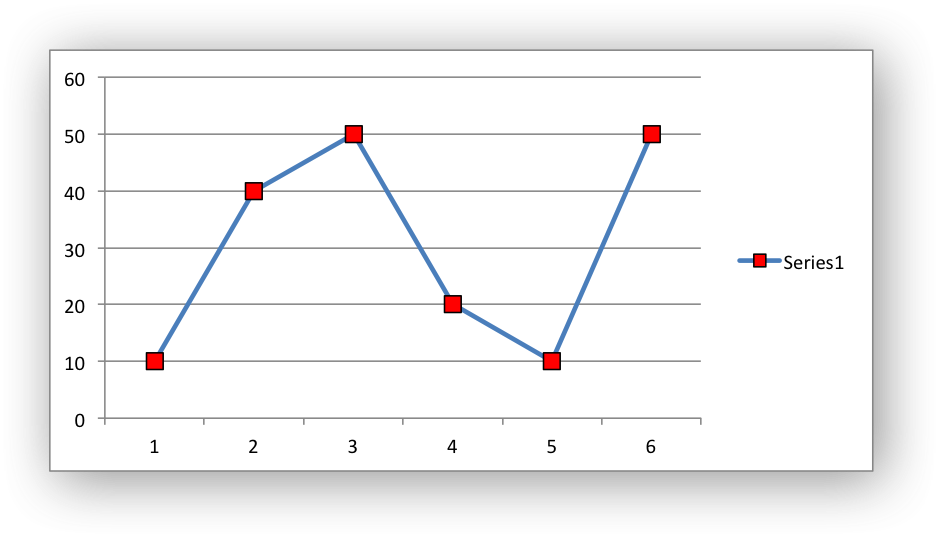For more information on line/border and fill formatting see Chart formatting: Line and Chart formatting: Fill below.

# Working with Chart Trendlines

A trendline can be added to a chart series to indicate trends in the data such as a moving average or a polynomial fit. The trendlines types are shown in the following Excel dialog: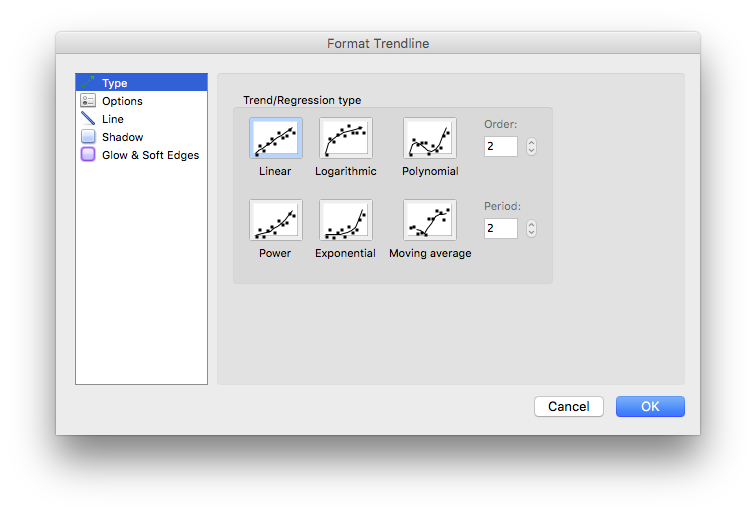The `chart_series_set_trendline()` function turns on these trendlines for a data series: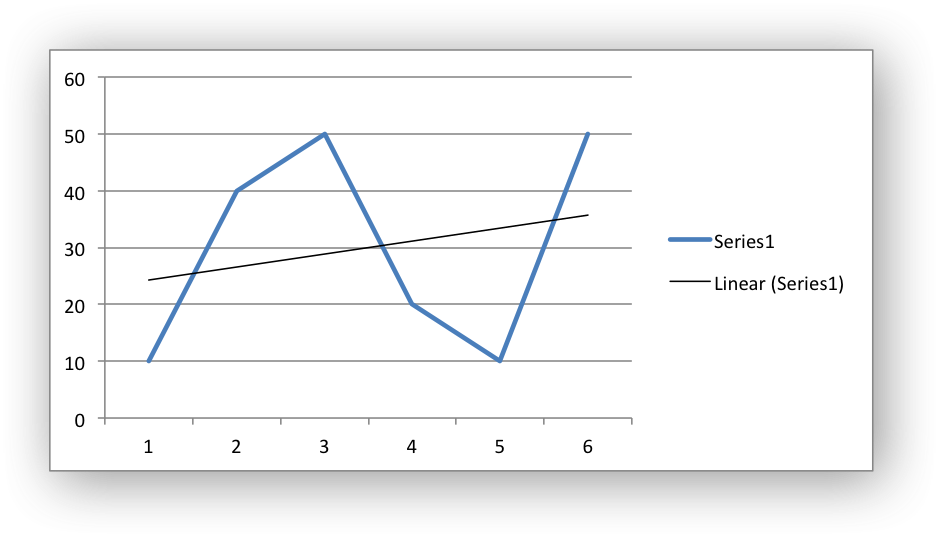The `value` parameter corresponds to order for a polynomial trendline and period for a Moving Average trendline. It both cases it must be >= 2. The `value` parameter is ignored for all other trendlines: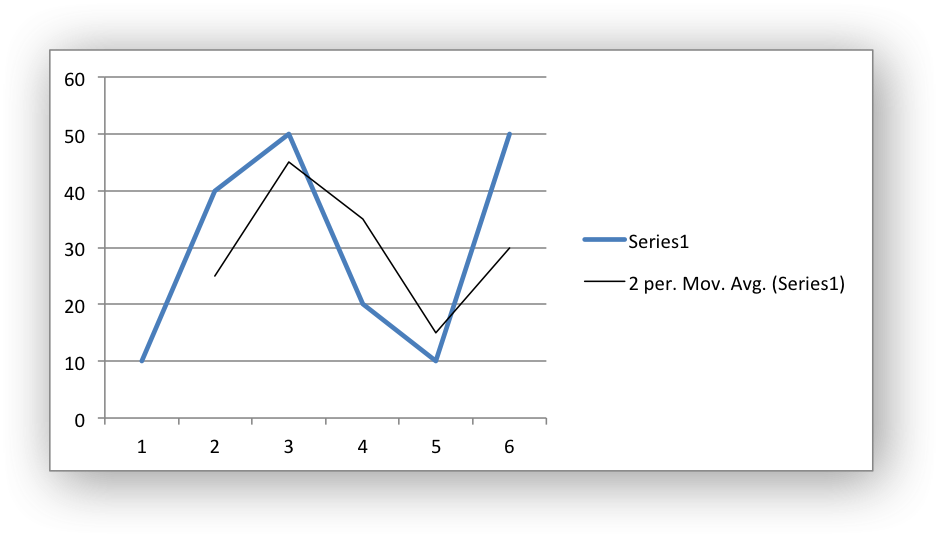The allowable values for the the trendline `type` are:

Other trendline options, such as those shown in the following Excel dialog, can be set using the functions described below.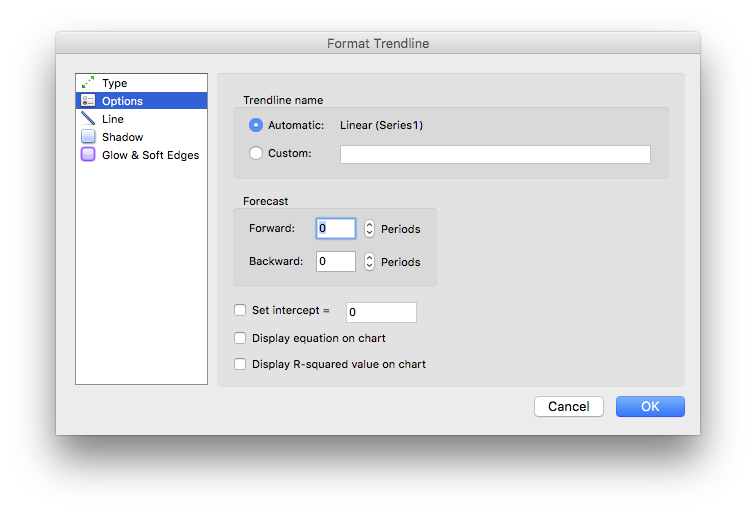The `chart_series_set_trendline_forecast()` function sets the forward and backward forecast periods for the trendline: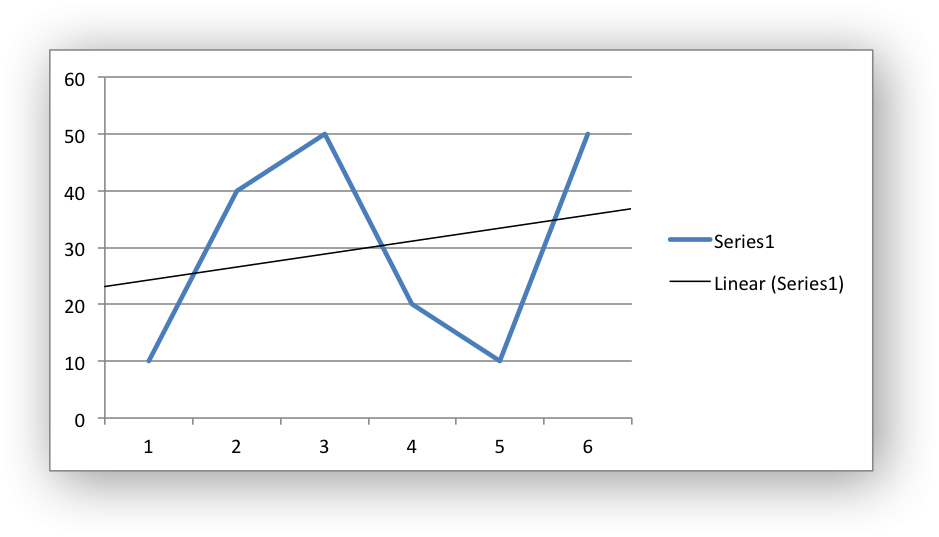Note
This feature isn't available for Moving Average in Excel.

The `chart_series_set_trendline_equation()` function displays the equation of the trendline on the chart: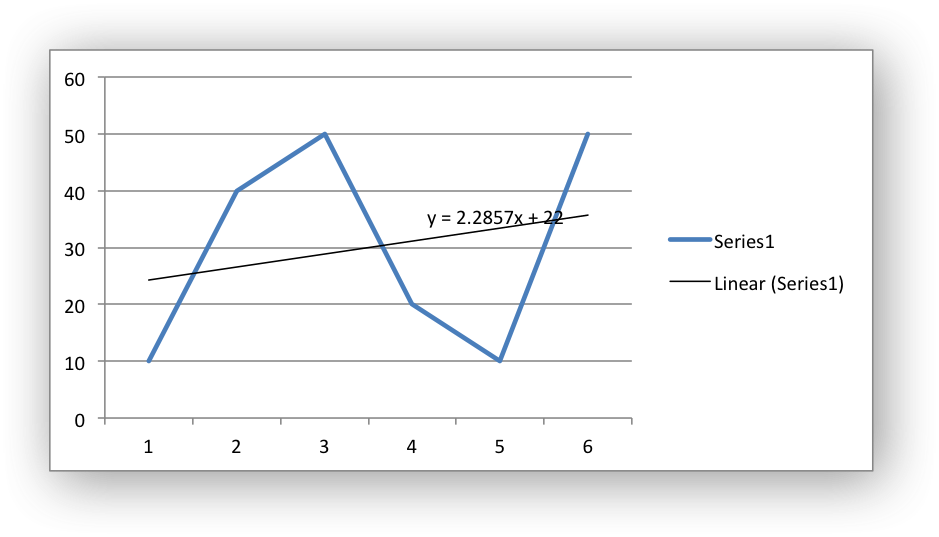Note
This feature isn't available for Moving Average in Excel.

The `chart_series_set_trendline_r_squared()` function displays the R-squared value for the trendline on the chart: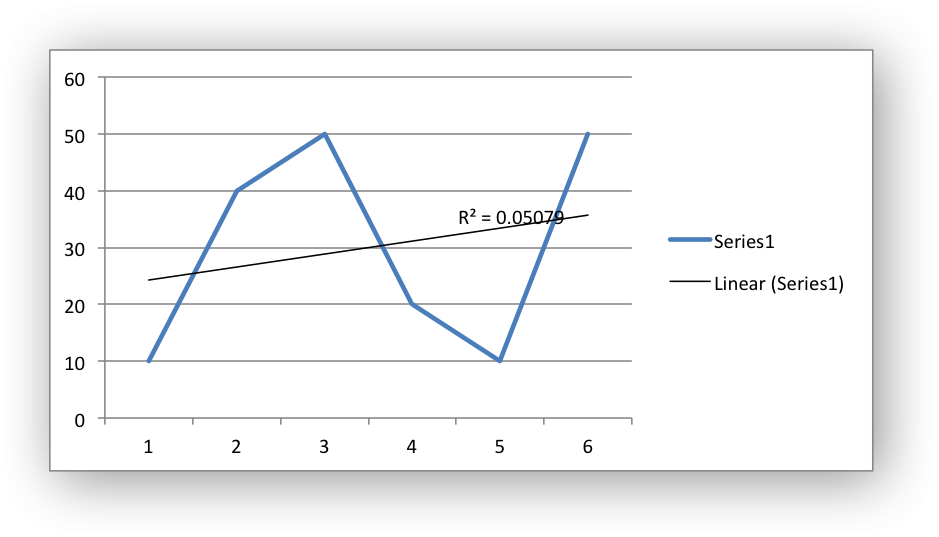Note
This feature isn't available for Moving Average in Excel.

The `chart_series_set_trendline_intercept()` function sets the Y-axis intercept for the trendline: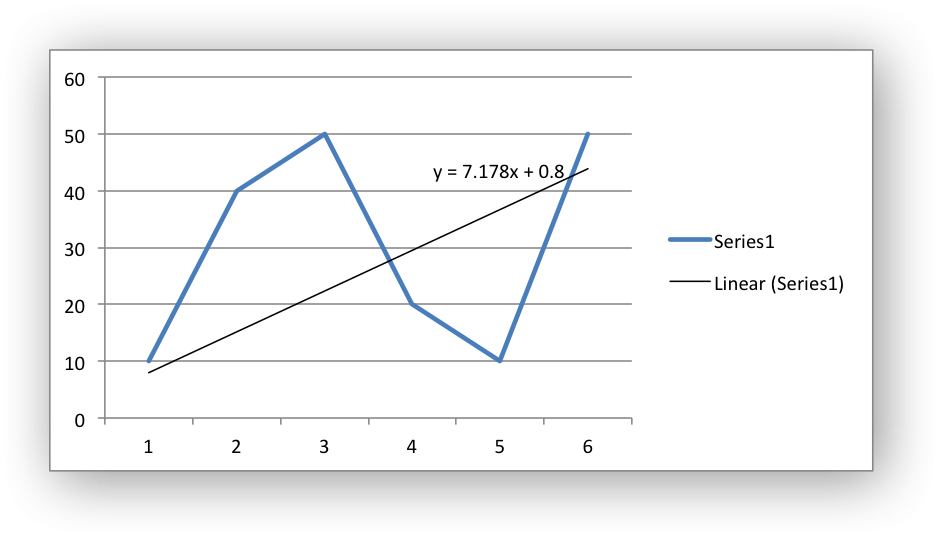As can be seen from the equation on the chart the intercept point (when X=0) is the same as the value set in the equation.

Note
The intercept feature is only available in Excel for Exponential, Linear and Polynomial trendline types.

The `chart_series_set_trendline_name()` function sets the name of the trendline that is displayed in the chart legend. In the examples above the trendlines are displayed with default names like "Linear (Series 1)" and "2 per Mov. Avg. (Series 1)". If these names are too verbose or not descriptive enough you can set your own trendline name:

chart_series_set_trendline_name(series, "My trendline");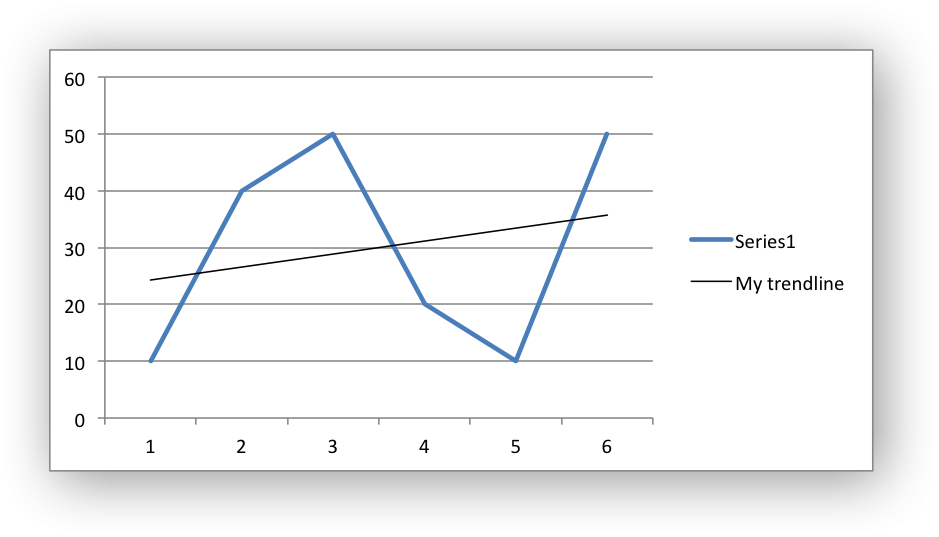It is often preferable to turn off the trendline caption in the legend. This is done in Excel by deleting the trendline name from the legend. In libxlsxwriter this is done using the `chart_legend_delete_series()` function to delete the zero based series numbers:

// Delete the series name for the second series (=1 in zero base).
// The -1 value indicates the end of the array of values.
int16_t names[] = {1, -1};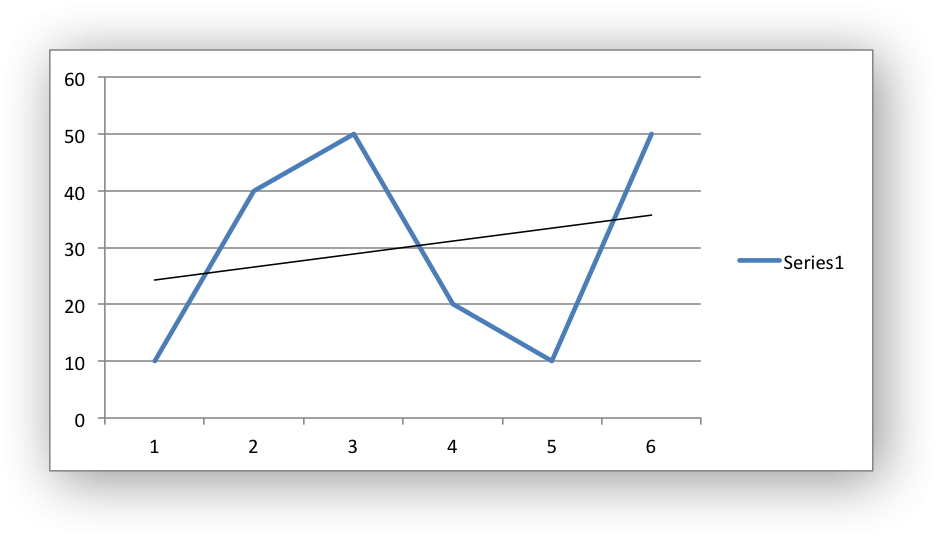The `chart_series_set_trendline_line()` function is used to set the line properties of a trendline: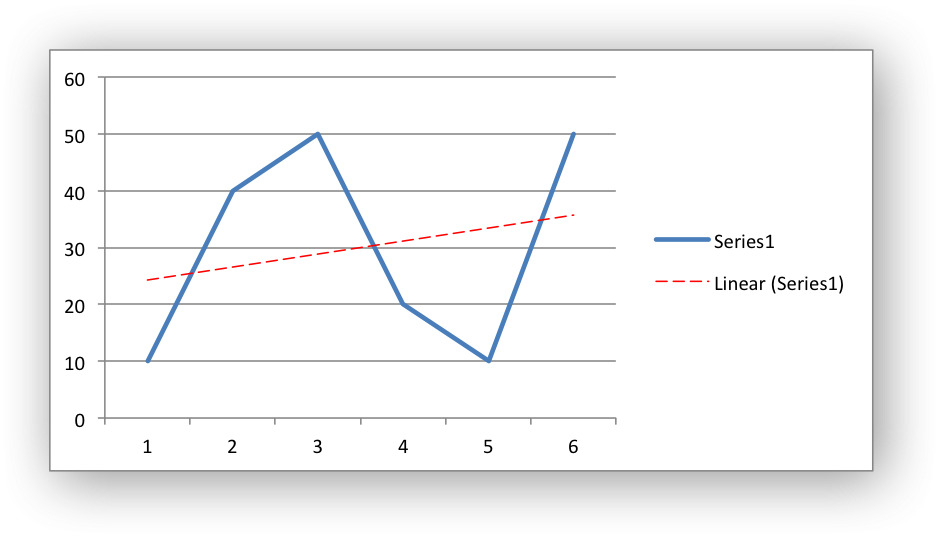Note
Trendlines cannot be added to series in a stacked chart, pie chart, doughnut chart or radar chart.

# Working with Chart Error Bars

Error bars can be added to a chart series to indicate error bounds in the data.

The error bars can be vertical `y_error_bars` (the most common type) or horizontal `x_error_bars` (for Bar and Scatter charts only). For convenience these can be accessed from a series as follows:

chart_series_set_error_bars(series->y_error_bars, ...);
chart_series_set_error_bars(series->x_error_bars, ...);

The error bar properties that can be set in Excel are show in the following dialog: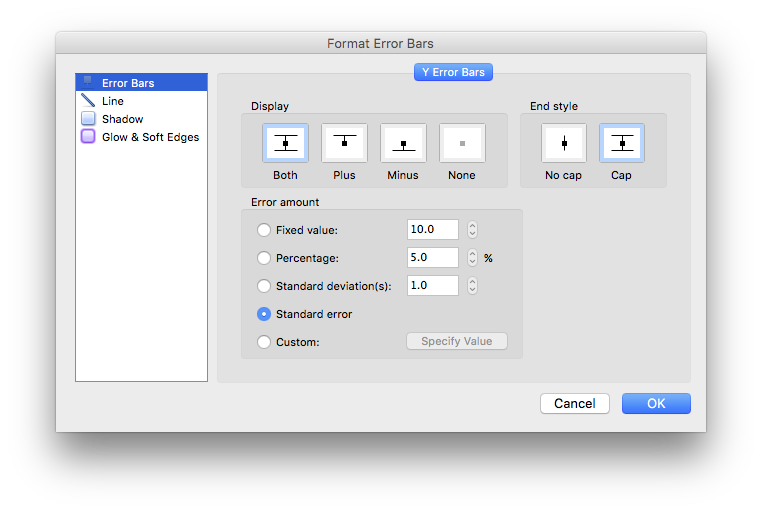Some of these properties can be set using the functions discussed below.

The `chart_series_set_error_bars()` function sets the error bar type and value associated with the type:

"=Sheet1!\$A\$1:\$A\$5",
"=Sheet1!\$B\$1:\$B\$5");
chart_series_set_error_bars(series->y_error_bars,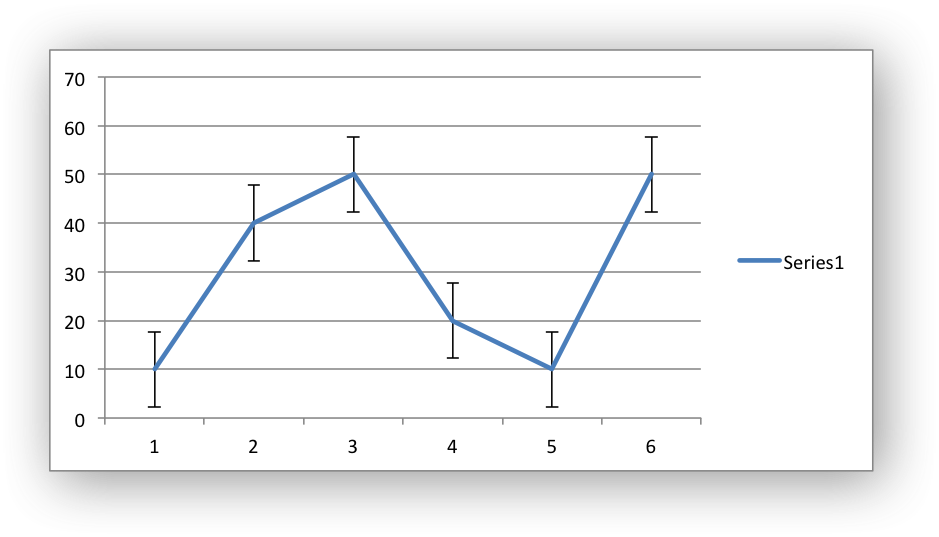The error bar types that be used are:

Note
Custom error bars are not currently supported.

All error bar types, apart from Standard error, should have a valid value to set the error range:

chart_series_set_error_bars(series1->y_error_bars,
chart_series_set_error_bars(series2->y_error_bars,
chart_series_set_error_bars(series3->y_error_bars,

For the Standard error type the value is ignored.

The `chart_series_set_error_bars_direction()` function sets the direction of the error bars:

chart_series_set_error_bars(series->y_error_bars,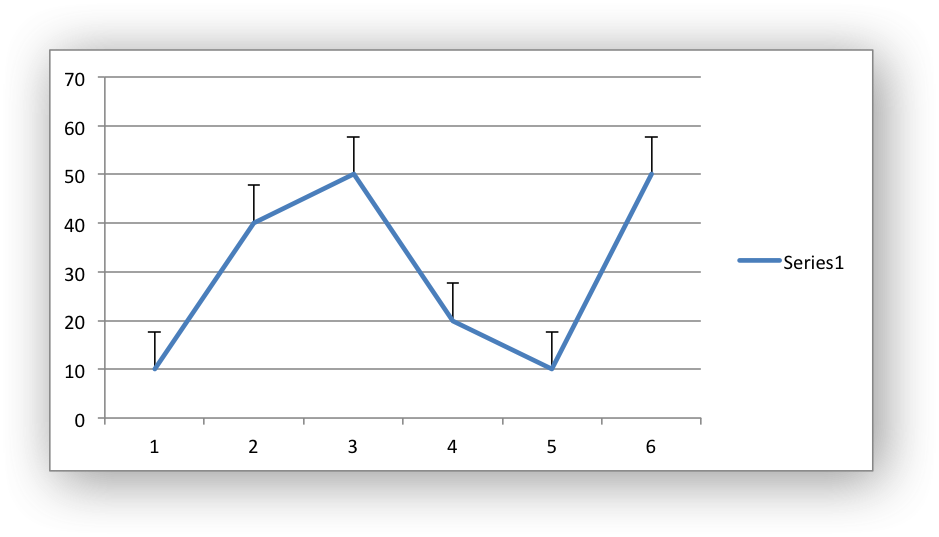The valid directions are:

The `chart_series_set_error_bars_endcap()` function sets the end cap type for the error bars:

chart_series_set_error_bars(series->y_error_bars,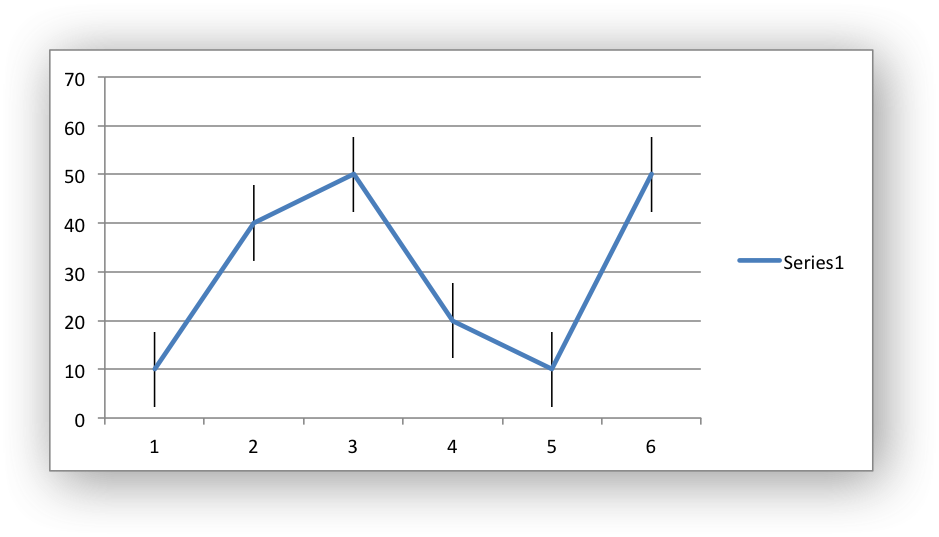The valid values are:

The `chart_series_set_error_bars_line()` function sets the line properties for the error bars:

chart_series_set_error_bars(series->y_error_bars,
chart_series_set_error_bars_line(series->y_error_bars, &line);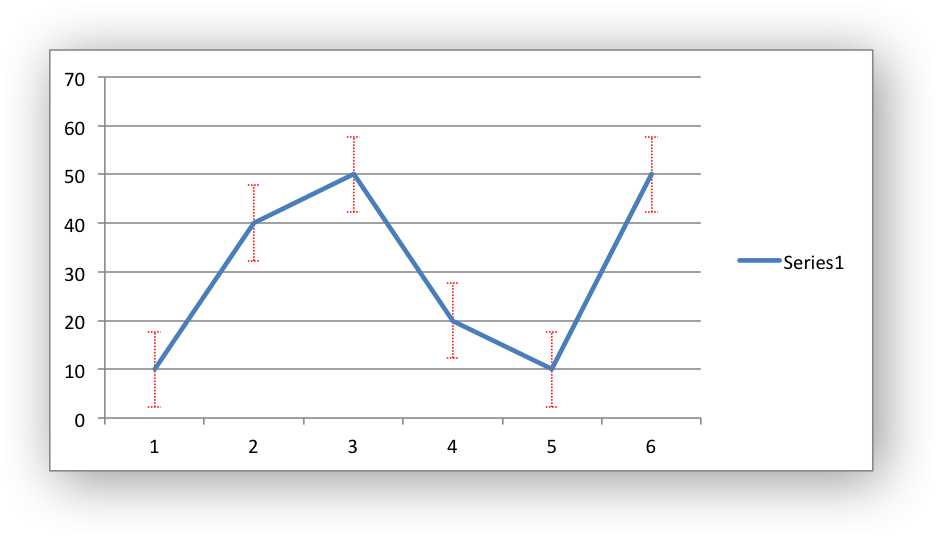# Working with Chart Points

In general formatting is applied to an entire series in a chart. However, it is occasionally required to format individual points within a series.

In Excel charts "points" have a different meaning depending on the type of chart:

• Line/Scatter chart: points are used to reference individual markers.
• Bar/Column/Area charts: points are used to reference individual bars or areas.
• Pie/Doughnut charts: points are used to reference each segment.

The most common use case is to format segments of a pie chart like this example:

#include "xlsxwriter.h"
/*
* Create a worksheet with an example Pie chart.
*/
int main() {
lxw_workbook *workbook = workbook_new("chart_pie_colors.xlsx");
/* Write some data for the chart. */
worksheet_write_string(worksheet, CELL("A1"), "Pass", NULL);
worksheet_write_string(worksheet, CELL("A2"), "Fail", NULL);
worksheet_write_number(worksheet, CELL("B1"), 90, NULL);
worksheet_write_number(worksheet, CELL("B2"), 10, NULL);
/* Create a pie chart. */
/* Add the data series to the chart. */
"=Sheet1!\$B\$1:\$B\$2");
/* Create some fills for the chart points/segments. */
/* Add the fills to the point objects. */
lxw_chart_point red_point = {.fill = &red_fill };
lxw_chart_point green_point = {.fill = &green_fill};
/* Create an array of pointer to chart points. Note, the array should be
* NULL terminated. */
lxw_chart_point *points[] = {&green_point,
&red_point,
NULL};
/* Add the points to the series. */
chart_series_set_points(series, points);
/* Insert the chart into the worksheet. */
worksheet_insert_chart(worksheet, CELL("D2"), chart);
return workbook_close(workbook);
}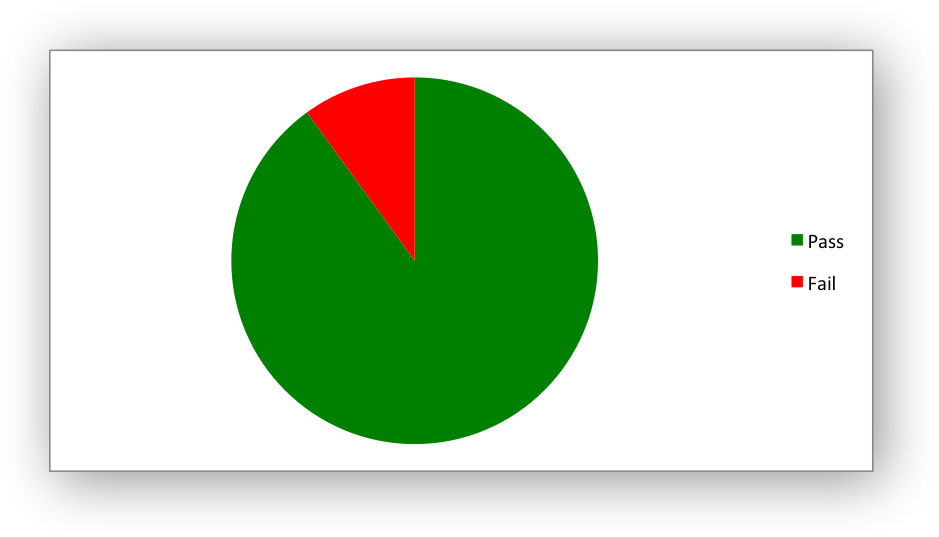The lxw_chart_point objects can be used to set the following properties for a chart point:

• Line/Border
• Fill
• Pattern

These properties are explained in the Chart Formatting subsections below.

The points should be passed as a NULL terminated array of pointers to lxw_chart_point objects:

lxw_chart_point red_point = {.fill = &red_fill };
lxw_chart_point green_point = {.fill = &green_fill};
lxw_chart_point *points[] = {&green_point,
&red_point,
NULL}; // Indicates the end of the list.
chart_series_set_points(series, points);

You can skip points in the series that you don't want to format by passing a zero-initialized lxw_chart_point:

lxw_chart_point default_point = {0, 0, 0};
lxw_chart_point red_point = {.fill = &red_fill, .line = &line};
lxw_chart_point green_point = {.fill = &green_fill, .line = &line};
lxw_chart_point *points[] = {&red_point,
&default_point,
&green_point,
&default_point,
&red_point,
NULL};
chart_series_set_points(series, points);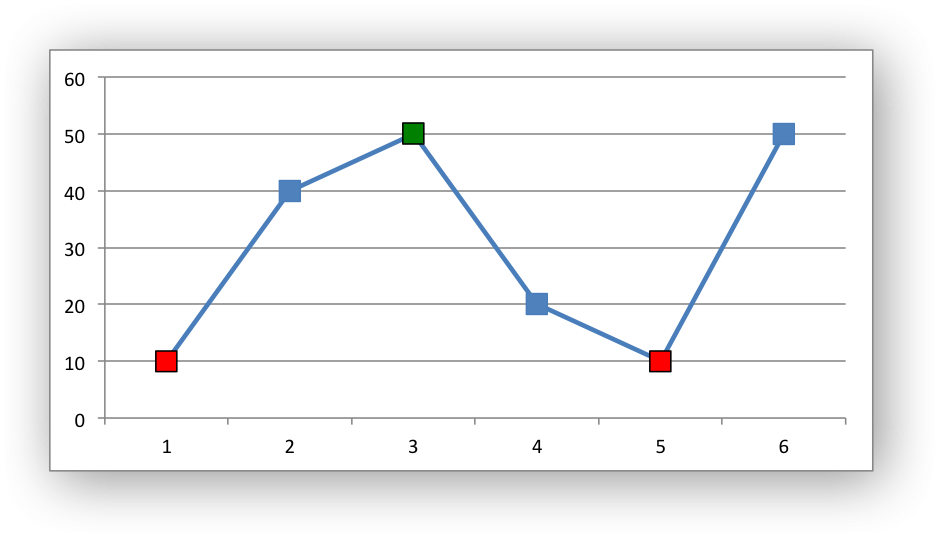The array of lxw_chart_point objects pointers corresponds to the order of the points in the series starting from the first point. However, it does not have to extend to the entire range of the series. It can be NULL terminated at any point in the series, such as in the previous example.

For Bar/Column/Area charts "points" refer to bars/areas within the chart:

lxw_chart_point red_point = {.fill = &red_fill};
lxw_chart_point default_point = {0, 0, 0};
lxw_chart_point *points[] = {&default_point, &default_point, &red_point, NULL};
chart_series_set_points(series2, points);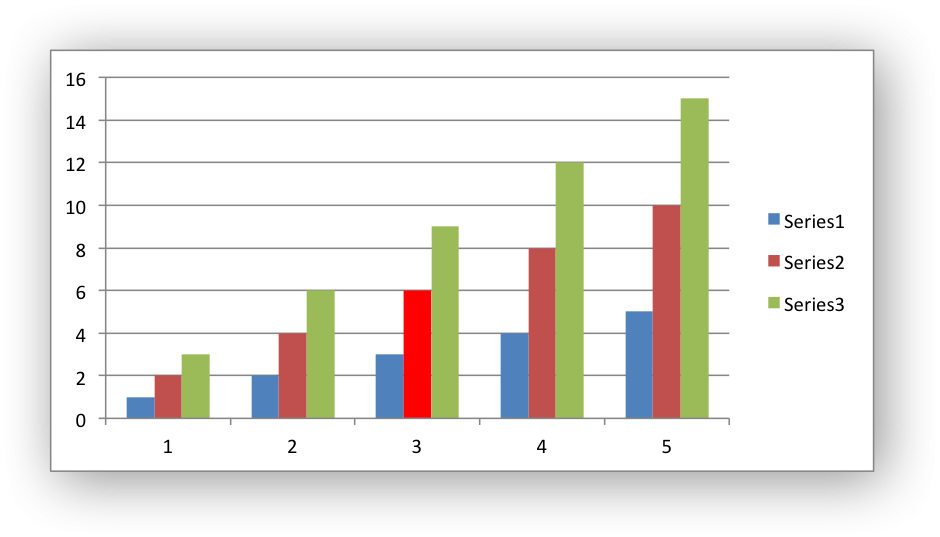# Working with Chart Data Labels

Data labels can be added to a chart series to indicate the values of the plotted data points. The functions described below turn on and set data label properties.

The `chart_series_set_labels()` function is used to turn on data labels for a chart series: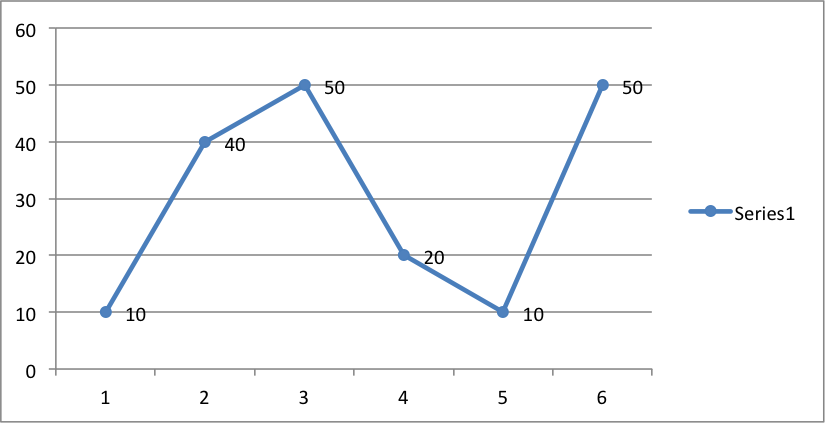By default data labels are displayed in Excel with only the values shown: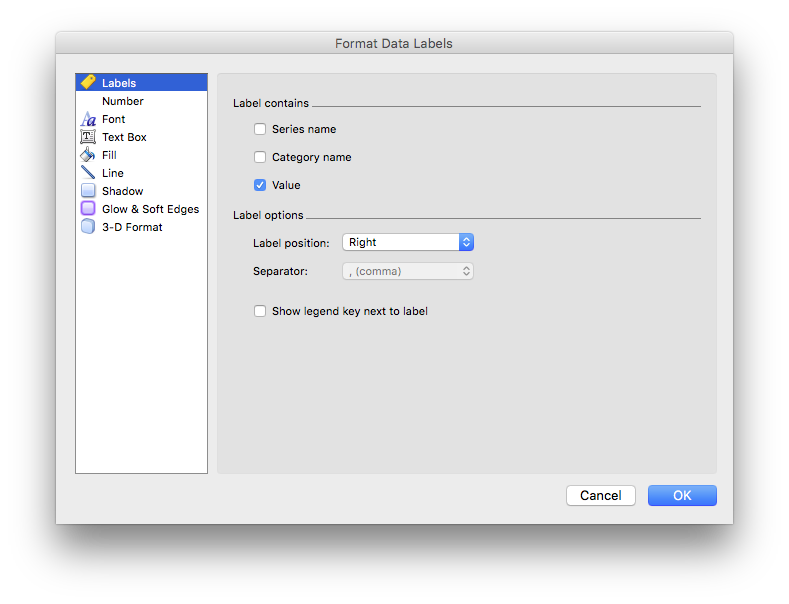However, it is possible to configure other display options, as shown in the functions below.

The `chart_series_set_labels_options()` function is used to set the parameters that are displayed in the series data label: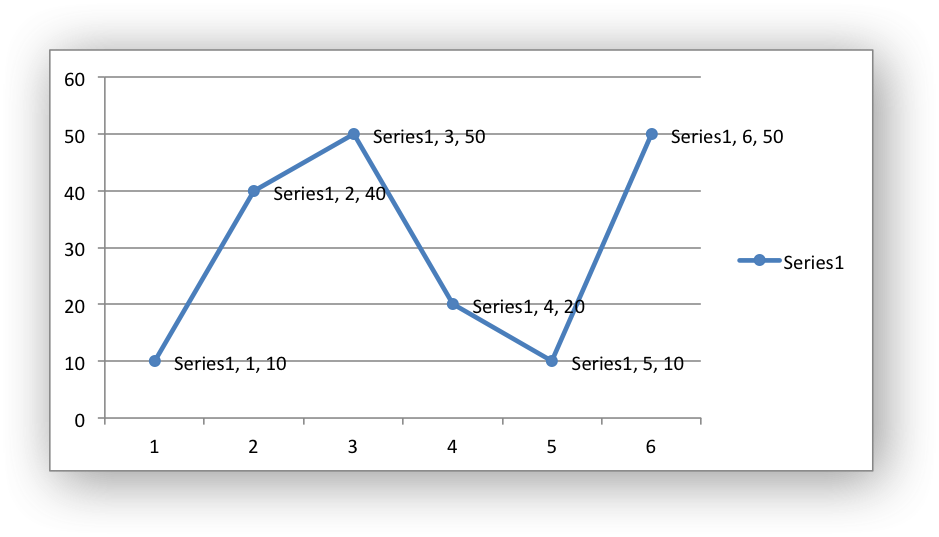The `chart_series_set_labels_separator()` function is used to change the separator between multiple data label items. The default options is a comma separator as shown in the previous example.

The available options are:

For example: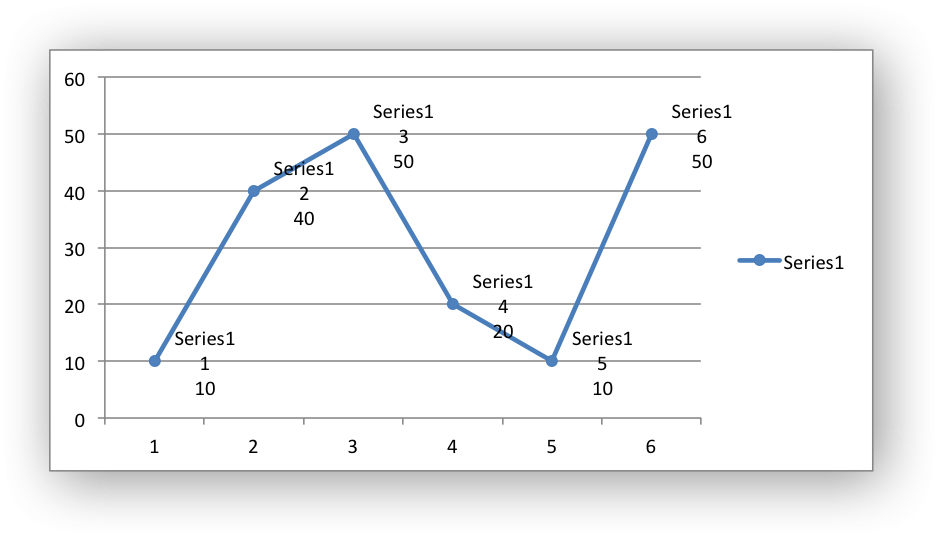The `chart_series_set_labels_position()` function sets the position of the labels in the data series: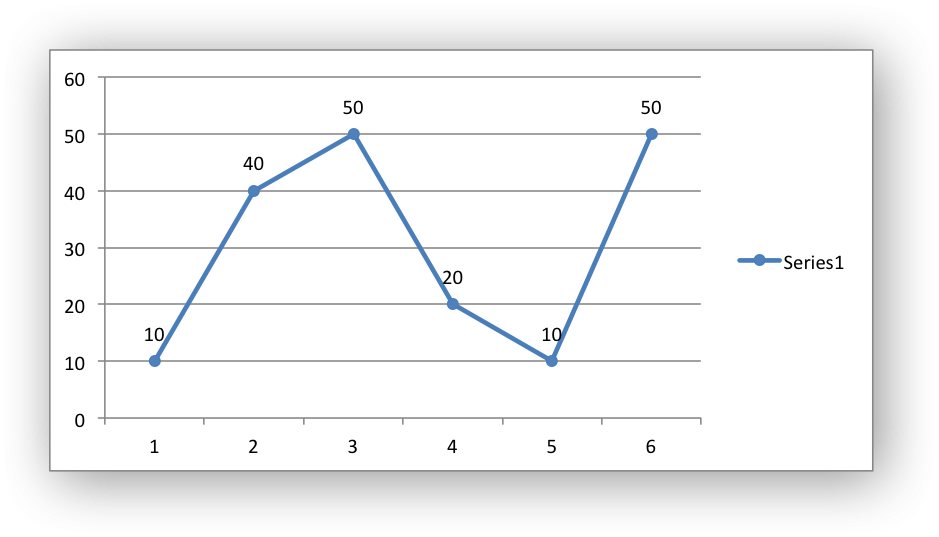In Excel the allowable data label positions vary for different chart types. The allowable, and default, positions are:

Position Line, Scatter Bar, Column Pie, Doughnut Area, Radar
LXW_CHART_LABEL_POSITION_CENTER Yes Yes Yes Yes (default)
LXW_CHART_LABEL_POSITION_RIGHT Yes (default)
LXW_CHART_LABEL_POSITION_LEFT Yes
LXW_CHART_LABEL_POSITION_ABOVE Yes
LXW_CHART_LABEL_POSITION_BELOW Yes
LXW_CHART_LABEL_POSITION_INSIDE_BASE Yes
LXW_CHART_LABEL_POSITION_INSIDE_END Yes Yes
LXW_CHART_LABEL_POSITION_OUTSIDE_END Yes (default) Yes
LXW_CHART_LABEL_POSITION_BEST_FIT Yes (default)

The `chart_series_set_labels_leader_line()` function is used to turn on leader lines for the data label of a series. It is mainly used for pie or doughnut charts:

Note
Even when leader lines are turned on they aren't automatically visible in Excel or XlsxWriter. Due to an Excel limitation (or design) leader lines only appear if the data label is moved manually or if the data labels are very close and need to be adjusted automatically.

The `chart_series_set_labels_legend()` function is used to set the legend key for a data series: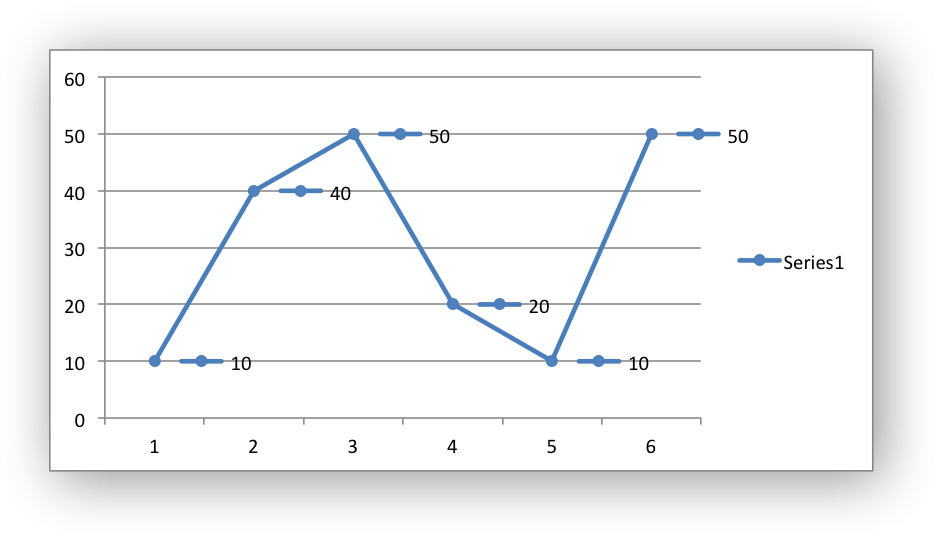The `chart_series_set_labels_percentage()` function is used to turn on the display of data labels as a percentage for a series. It is mainly used for pie charts: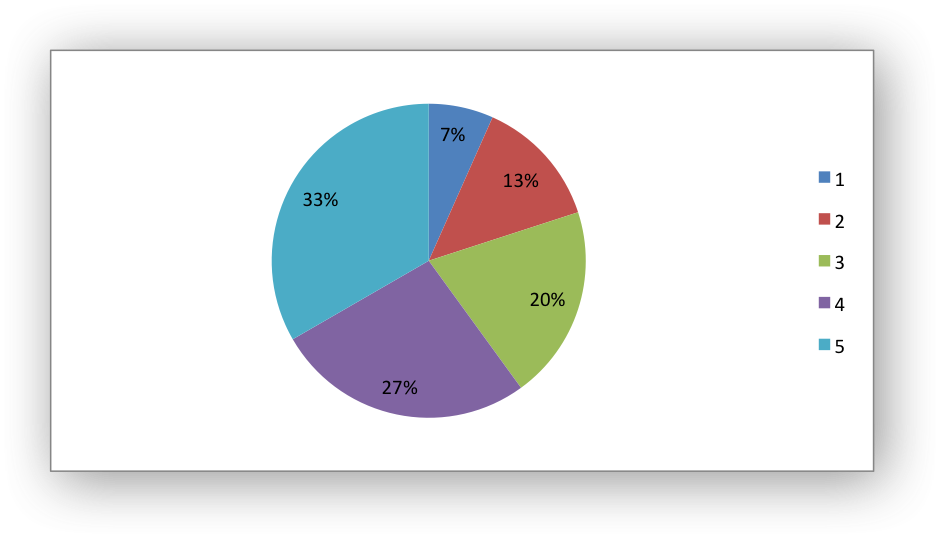The `chart_series_set_labels_num_format()` function is used to set the number format for data labels: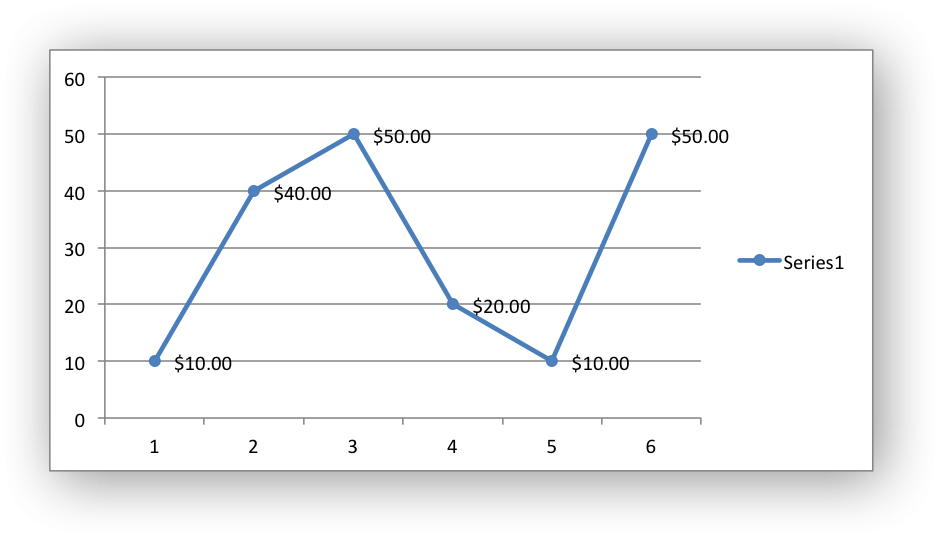The number format is similar to the Worksheet Cell Format num_format, see `format_set_num_format()`.

The `chart_series_set_labels_font()` function is used to set the font for data labels:

lxw_chart_font font = {.name = "Consolas", .color = LXW_COLOR_RED};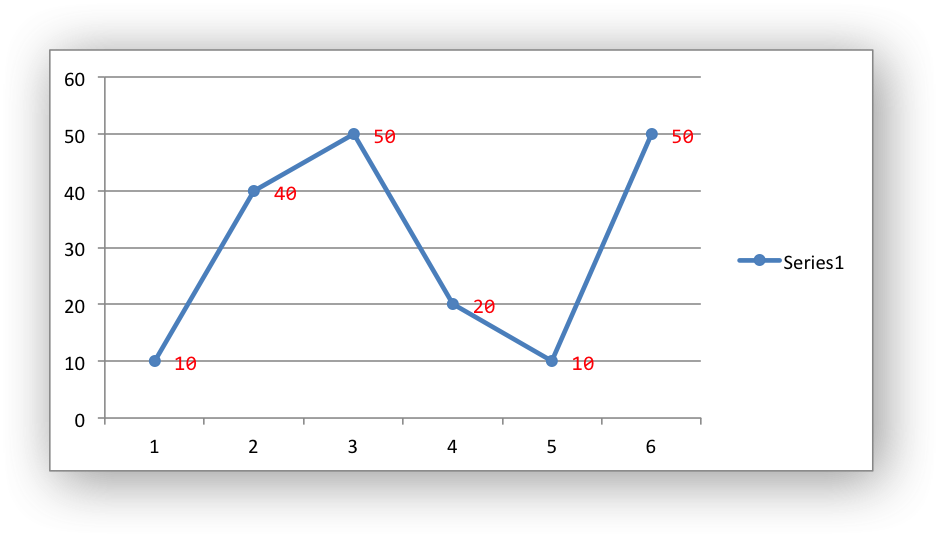The `chart_series_set_labels_line()`, `chart_series_set_labels_fill()` and `chart_series_set_labels_pattern()` functions can be used to set the formatting for a data series using standard chart formatting structs: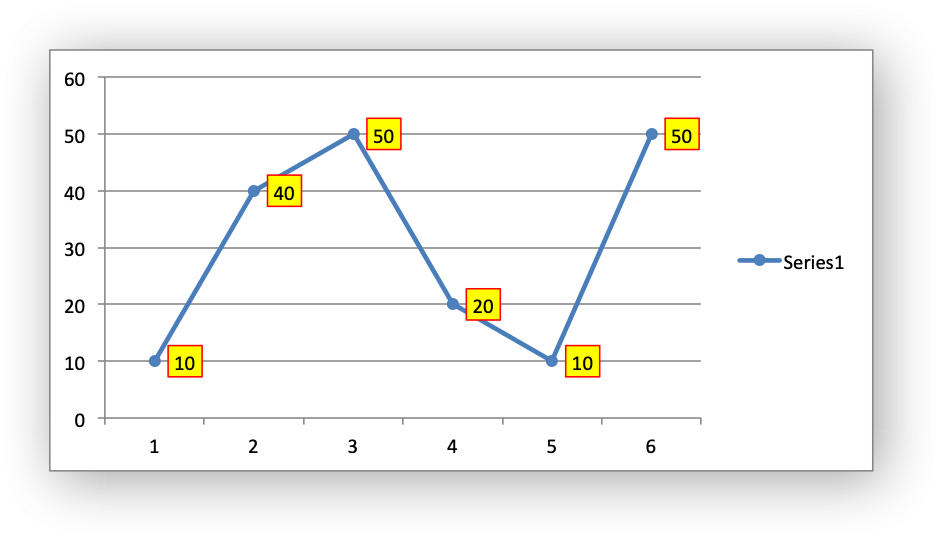## Custom Chart Data Labels

The `chart_series_set_labels_custom()` function is used to set the properties of individual data labels in a series. The most common use for this is to set custom text or number labels:

// Add the series data labels.
// Create some custom labels.
lxw_chart_data_label data_label1 = {.value = "Jan"};
lxw_chart_data_label data_label2 = {.value = "Feb"};
lxw_chart_data_label data_label3 = {.value = "Mar"};
lxw_chart_data_label data_label4 = {.value = "Apr"};
lxw_chart_data_label data_label5 = {.value = "May"};
lxw_chart_data_label data_label6 = {.value = "Jun"};
// Create an array of label pointers. NULL indicates the end of the array.
lxw_chart_data_label *data_labels[] = {
&data_label1,
&data_label2,
&data_label3,
&data_label4,
&data_label5,
&data_label6,
NULL
};
// Set the custom labels.
chart_series_set_labels_custom(series, data_labels);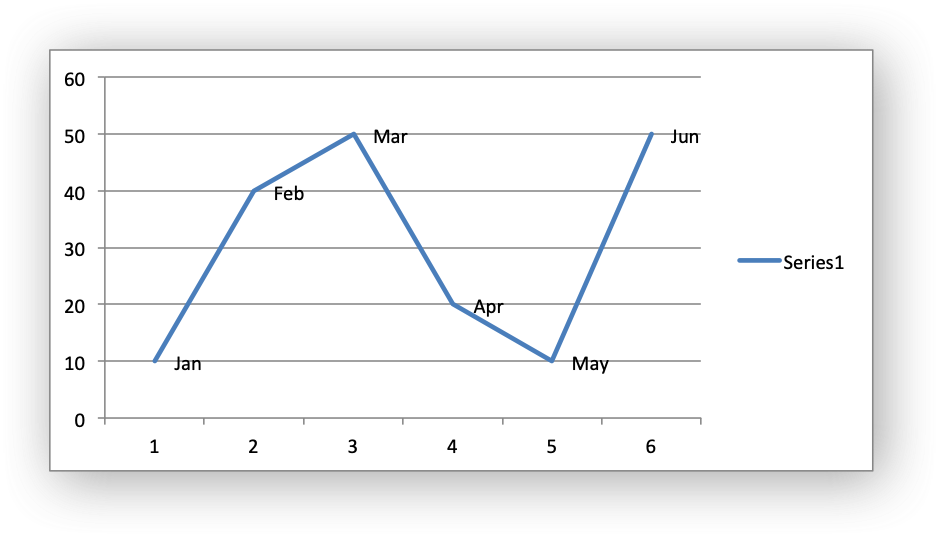As shown in the previous example the `chart_series_set_labels_custom()` function takes a pointer to an array of lxw_chart_data_label pointers. The list should be `NULL` terminated. Any lxw_chart_data_label items set to a default initialization or omitted from the list will be assigned the default data label value:

// Add the series data labels.
// Create some custom labels.
lxw_chart_data_label default_label = {0};
lxw_chart_data_label data_label2 = {.value = "Feb"};
lxw_chart_data_label data_label3 = {.value = "Mar"};
lxw_chart_data_label data_label4 = {.value = "Apr"};
// Create an array of label pointers. NULL indicates the end of the array.
lxw_chart_data_label *data_labels[] = {
&default_label,
&data_label2,
&data_label3,
&data_label4,
NULL
};
// Set the custom labels.
chart_series_set_labels_custom(series, data_labels);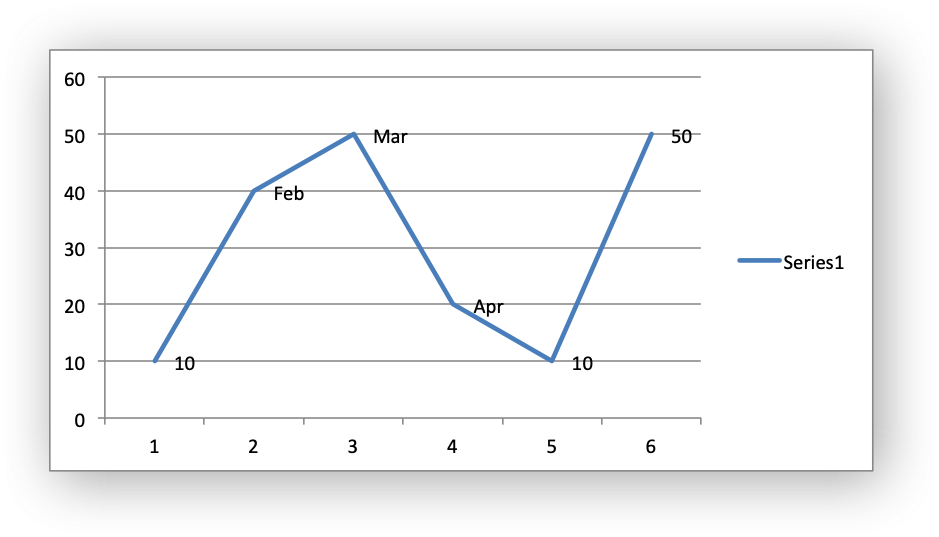The `value` element in the lxw_chart_data_label struct should be a string, a number as a string, or formula string that refers to a cell from which the value will be taken:

// Add the series data labels.
// Create some custom labels.
lxw_chart_data_label data_label1 = {.value = "=Sheet1!\$C\$1"};
lxw_chart_data_label data_label2 = {.value = "=Sheet1!\$C\$2"};
lxw_chart_data_label data_label3 = {.value = "=Sheet1!\$C\$3"};
lxw_chart_data_label data_label4 = {.value = "=Sheet1!\$C\$4"};
lxw_chart_data_label data_label5 = {.value = "=Sheet1!\$C\$5"};
lxw_chart_data_label data_label6 = {.value = "=Sheet1!\$C\$6"};
// Create an array of label pointers. NULL indicates the end of the array.
lxw_chart_data_label *data_labels[] = {
&data_label1,
&data_label2,
&data_label3,
&data_label4,
&data_label5,
&data_label6,
NULL
};
// Set the custom labels.
chart_series_set_labels_custom(series, data_labels);

The `font` element in the lxw_chart_data_label struct can be used to set the font for the label in the data series (see Chart formatting: Fonts):

// Add the series data labels.
// Create some custom labels.
lxw_chart_data_label data_label1 = {.value = "=Sheet1!\$C\$1", .font = &font};
lxw_chart_data_label data_label2 = {.value = "=Sheet1!\$C\$2", .font = &font};
lxw_chart_data_label data_label3 = {.value = "=Sheet1!\$C\$3", .font = &font};
lxw_chart_data_label data_label4 = {.value = "=Sheet1!\$C\$4", .font = &font};
lxw_chart_data_label data_label5 = {.value = "=Sheet1!\$C\$5", .font = &font};
lxw_chart_data_label data_label6 = {.value = "=Sheet1!\$C\$6", .font = &font};
// Create an array of label pointers. NULL indicates the end of the array.
lxw_chart_data_label *data_labels[] = {
&data_label1,
&data_label2,
&data_label3,
&data_label4,
&data_label5,
&data_label6,
NULL
};
// Set the custom labels.
chart_series_set_labels_custom(series, data_labels);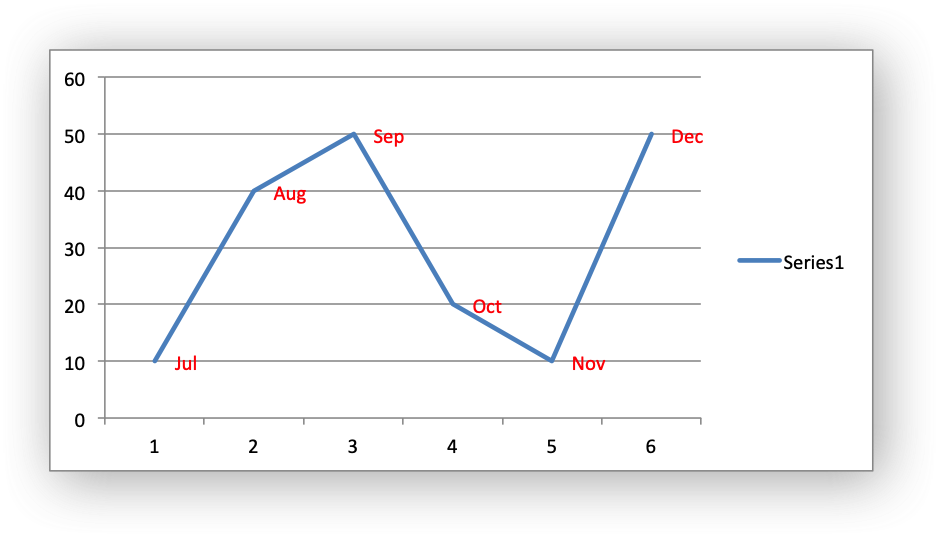The `hide` element in the lxw_chart_data_label struct can be used to hide/delete labels in a series. This can be useful if you want to highlight one or more cells in the series, for example the maximum and the minimum:

// Add the series data labels.
// Create some custom labels.
// Create an array of label pointers. NULL indicates the end of the array.
lxw_chart_data_label *data_labels[] = {
&hide,
&keep,
&hide,
&hide,
&keep,
&hide,
NULL
};
// Set the custom labels.
chart_series_set_labels_custom(series, data_labels);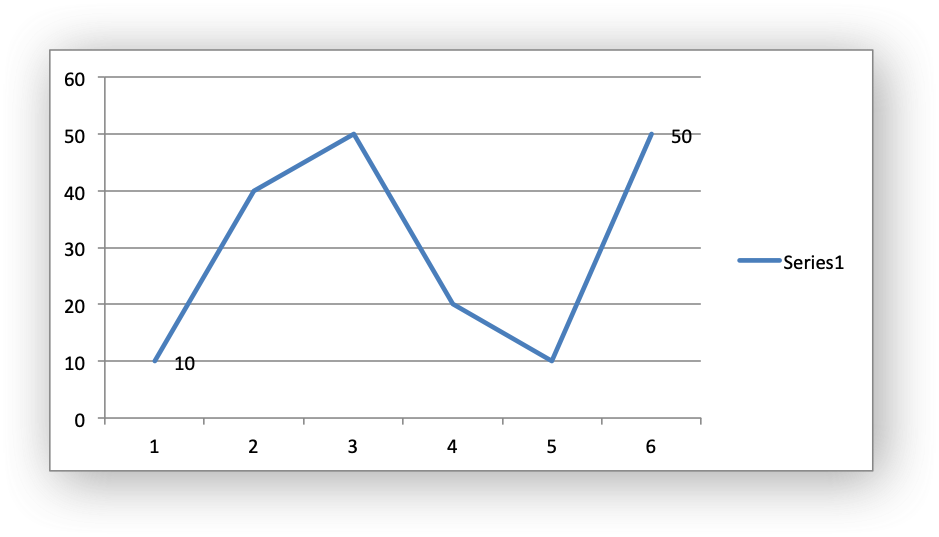Standard chart formatting such as lxw_chart_line, lxw_chart_fill and lxw_chart_pattern can also be applied to custom data labels via lxw_chart_data_label:

// Set the border/line and fill for the data labels.
// Create some custom labels.
lxw_chart_data_label data_label9_1 = {.value = "Amy", .line = &line3};
lxw_chart_data_label data_label9_2 = {.value = "Bea"};
lxw_chart_data_label data_label9_3 = {.value = "Eva"};
lxw_chart_data_label data_label9_4 = {.value = "Fay"};
lxw_chart_data_label data_label9_5 = {.value = "Liv"};
lxw_chart_data_label data_label9_6 = {.value = "Una", .fill = &fill3};
// Set the default formatting for the data labels in the series.
// Create an array of label pointers. NULL indicates the end of the array.
lxw_chart_data_label *data_labels9[] = {
&data_label9_1,
&data_label9_2,
&data_label9_3,
&data_label9_4,
&data_label9_5,
&data_label9_6,
NULL
};
/* Set the custom labels. */
chart_series_set_labels_custom(series, data_labels9);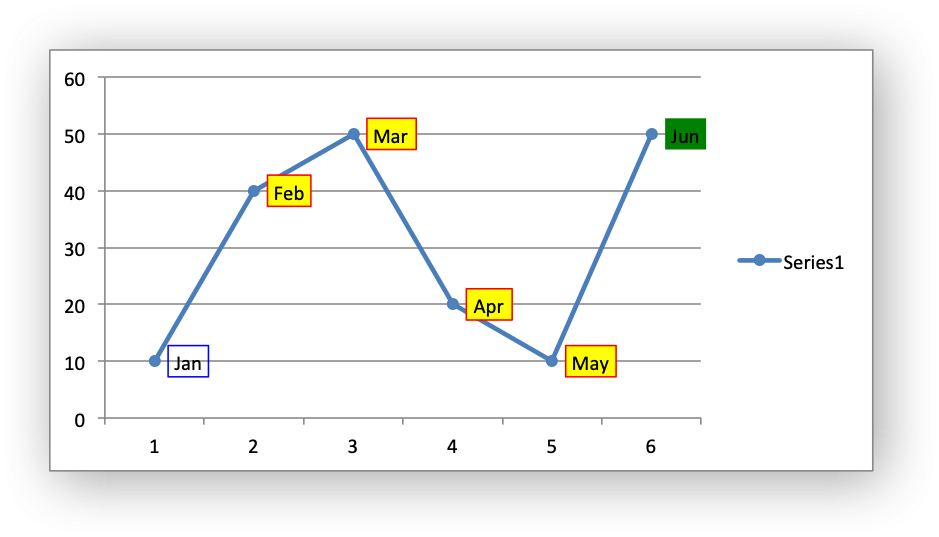# Chart Formatting

The following chart formatting properties can be set for any chart object that they apply to (and that are supported by libxlsxwriter) such as chart lines, column fill areas and other chart elements:

• Font
• Line/Border
• Fill
• Pattern

For example:

lxw_chart_line marker_line = {.color = LXW_COLOR_RED};
chart_series_set_line(series, &chart_line);
chart_series_set_marker_line(series, &marker_line);
chart_series_set_marker_fill(series, &marker_fill);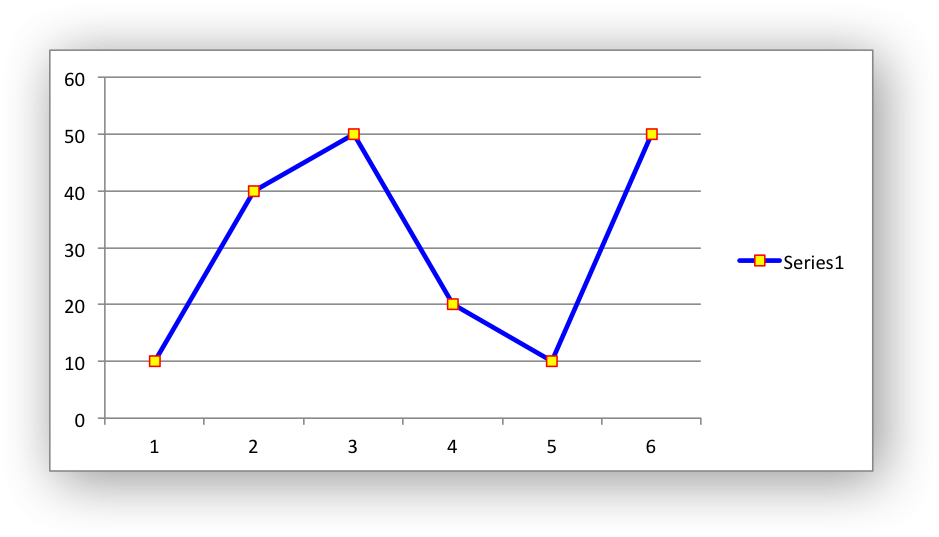These properties are explained in the subsections below.

## Chart formatting: Fonts

Font properties can be set for several chart objects such as chart titles, axis labels, and axis numbering.

A chart font lxw_chart_font struct with default properties is:

lxw_chart_font font = {.name = "Calibri",
.size = 10,
.bold = LXW_FALSE,
.italic = LXW_FALSE,
.underline = LXW_FALSE,
.rotation = 0,
.color = LXW_COLOR_BLACK};

The font properties are:

• `name`: Set the font name:
lxw_chart_font font = {.name = "Arial"};
• `size`: Set the font size:
lxw_chart_font font = {.name = "Arial", .size = 11};
• `bold`: Set the font bold property:
• `italic`: Set the font italic property:
• `underline`: Set the font underline property:
• `rotation`: Set the font rotation, angle, property in the range -90 to 90 deg:
lxw_chart_font font = {.rotation = 45};

This is useful for displaying axis data such as dates in a more compact format.

• `color`: Set the font color property. It can be a HTML style RGB color or a limited number of named colors (see Working with Colors for more information):

Here is a longer example with several chart formats:

lxw_chart_font font1 = {.name = "Calibri", .color = LXW_COLOR_BLUE};
lxw_chart_font font2 = {.name = "Courier", .color = 0x92D050};
lxw_chart_font font3 = {.name = "Arial", .color = 0x00B0F0};
lxw_chart_font font4 = {.name = "Century", .color = LXW_COLOR_RED};
lxw_chart_font font5 = {.rotation = -30};
.italic = LXW_TRUE,
.underline = LXW_TRUE,
.color = 0x7030A0};
/* Write the chart title with a font. */
chart_title_set_name(chart, "Test Results");
chart_title_set_name_font(chart, &font1);
/* Write the Y axis with a font. */
chart_axis_set_name(chart->y_axis, "Units");
/* Write the X axis with a font. */
chart_axis_set_name(chart->x_axis, "Month");
/* Display the chart legend at the bottom of the chart. */
chart_legend_set_font(chart, &font6);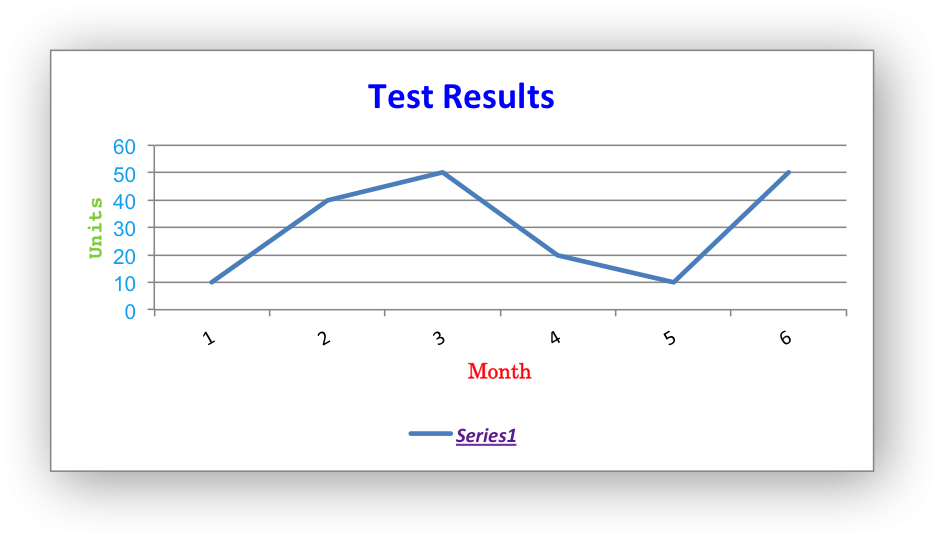## Chart formatting: Line

The line format is used to specify properties of line objects that appear in a chart such as a plotted line on a chart or a border.

A chart line lxw_chart_line struct with default properties is:

.color = LXW_COLOR_BLACK,
.width = 2.25,

The `none` member is used to turn the line off (it is always on by default except in Scatter charts). This is useful if you wish to plot a series with markers without a line, or a column fill without a border:

chart_series_set_line(series, &line);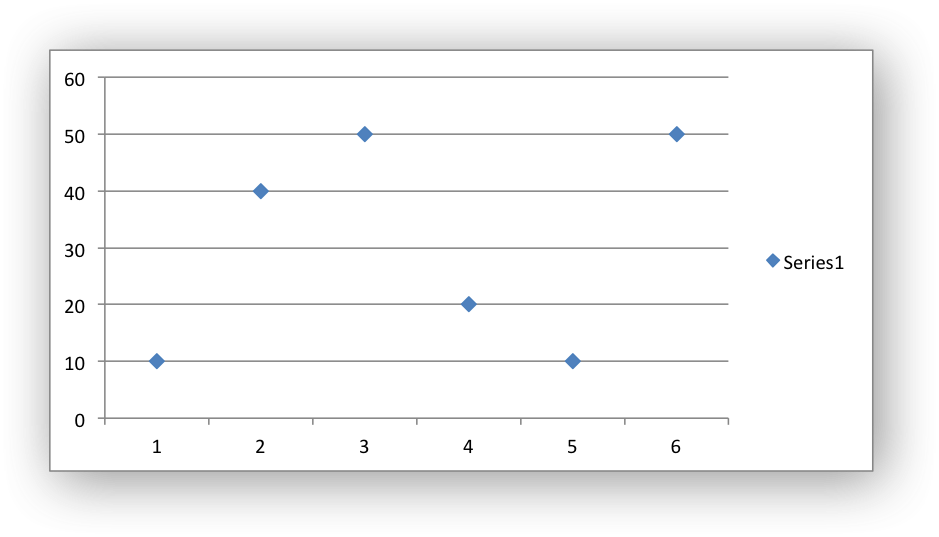The `color` member sets the color of the line. It can be a HTML style RGB color or a limited number of named colors (see Working with Colors for more information):

lxw_chart_line line = {.color = 0xFF9900};
chart_series_set_line(series, &line);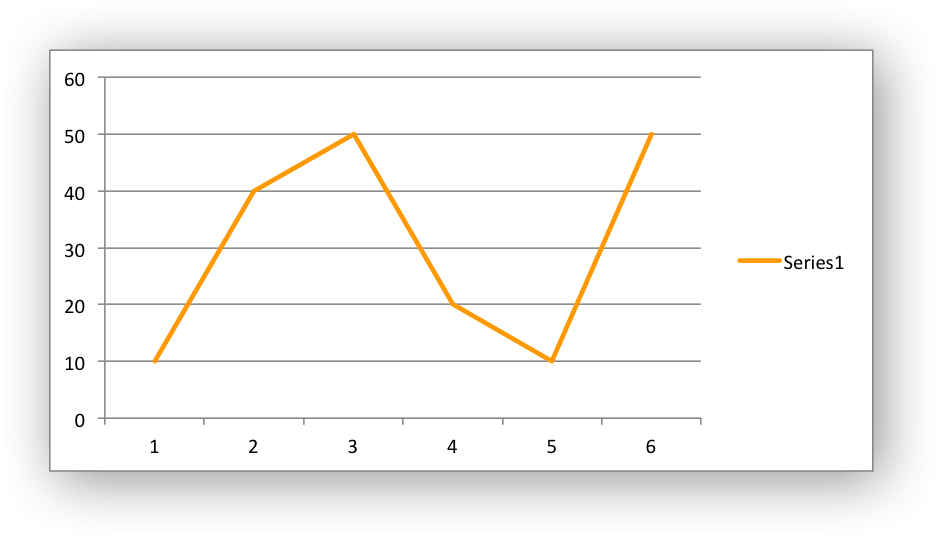The `width` member sets the width of the line. It should be specified in increments of 0.25 of a point as in Excel:

lxw_chart_line line = {.width = 4.25};
chart_series_set_line(series, &line);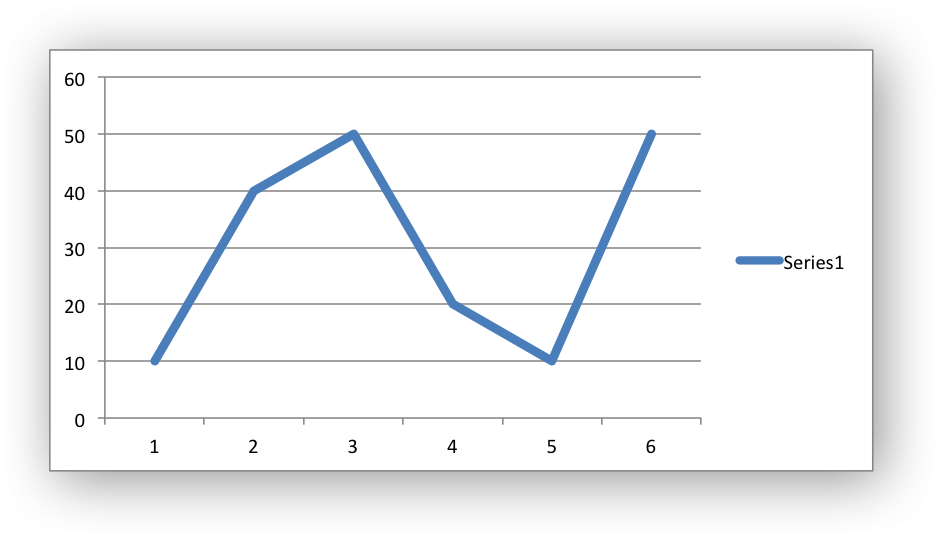The `dash_type` member sets the dash style of the line:

chart_series_set_line(series, &line);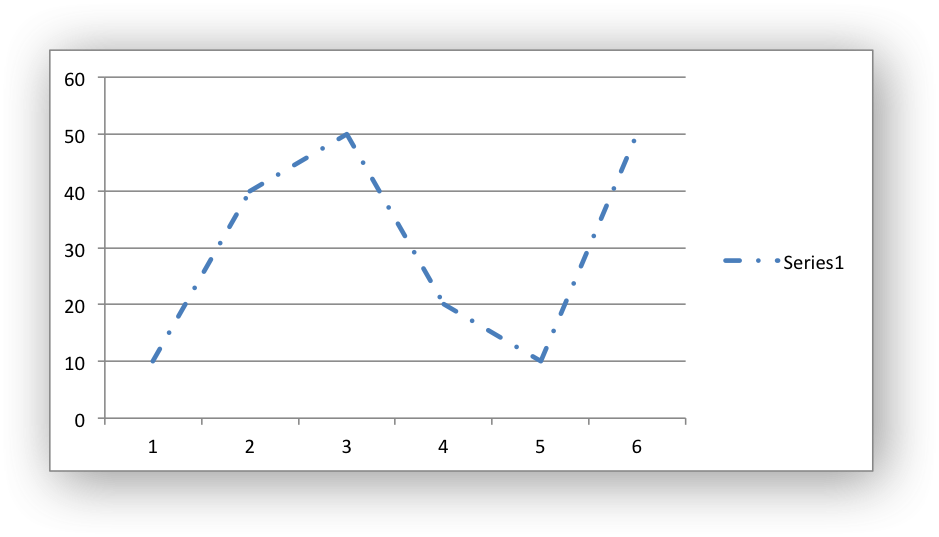The following lxw_chart_line_dash_type values are available. They are shown in the order that they appear in the Excel dialog:

Define Excel name
LXW_CHART_LINE_DASH_SOLID Solid
LXW_CHART_LINE_DASH_ROUND_DOT Round Dot
LXW_CHART_LINE_DASH_SQUARE_DOT Square Dot
LXW_CHART_LINE_DASH_DASH Dash
LXW_CHART_LINE_DASH_DASH_DOT Dash Dot
LXW_CHART_LINE_DASH_LONG_DASH Long Dash
LXW_CHART_LINE_DASH_LONG_DASH_DOT Long Dash Dot
LXW_CHART_LINE_DASH_LONG_DASH_DOT_DOT Long Dash Dot Dot

The default line style is `#LXW_CHART_LINE_DASH_SOLID`.

More than one line property can be specified at a time:

.width = 1.25,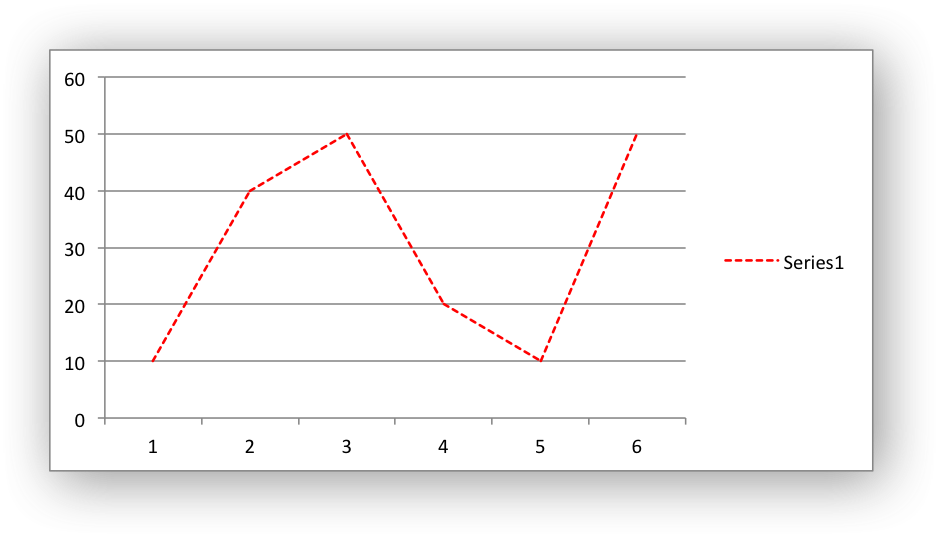## Chart formatting: Border

In Excel chart formatting the `border` property is a synonym for `line` when the object being formatting also has a `fill` property.

Anywhere you see `border` in an Excel chart dialog you can use the equivalent libxlsxwriter `line` function.

## Chart formatting: Fill

The fill format is used to specify properties of chart objects that internal boundaries such as a column chart.

A chart fill lxw_chart_fill struct with default properties is:

.color = LXW_COLOR_BLACK,
.transparency = 0};

The none property is used to turn the fill property off (it is generally on by default):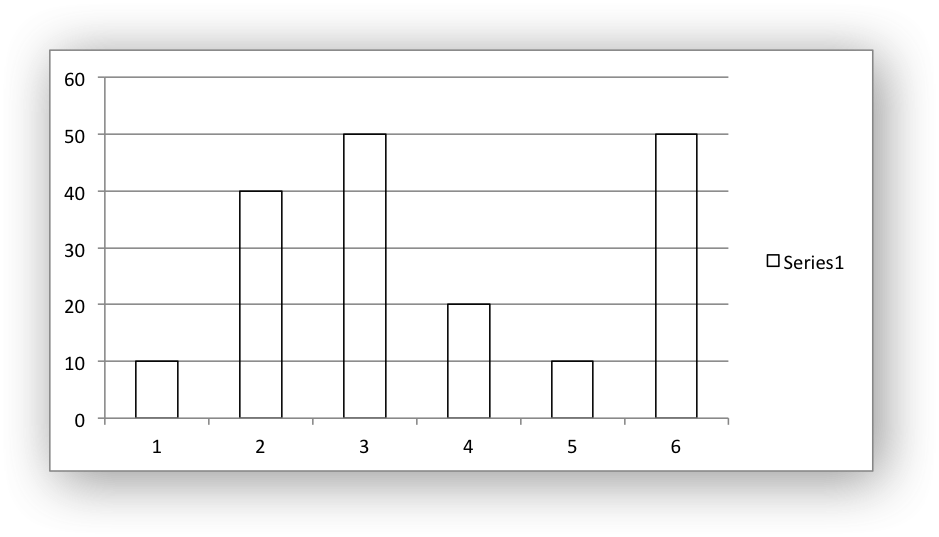The `color` member sets the color of the fill area. It can be a HTML style RGB color or a limited number of named colors (see Working with Colors for more information):

lxw_chart_fill fill = {.color = 0xFF9900};
chart_series_set_fill(series, &fill);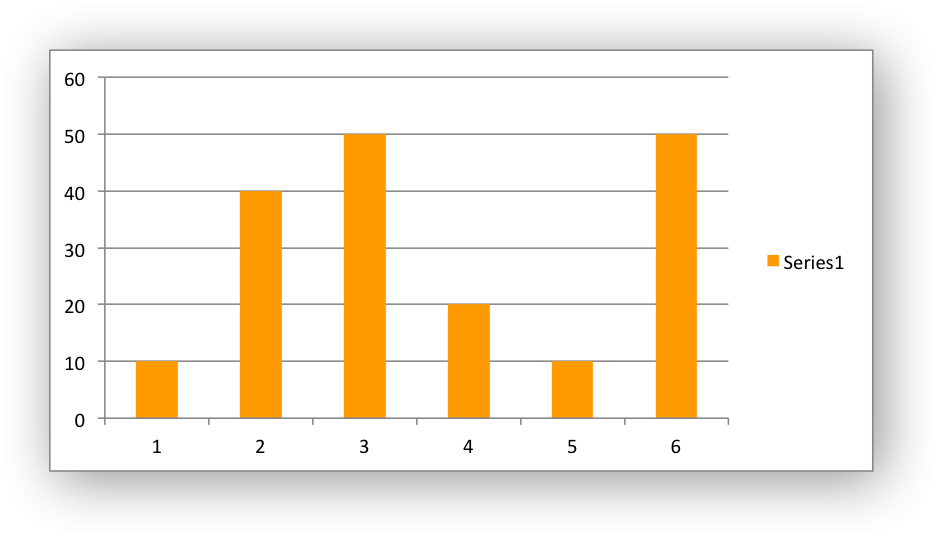The `transparency` member sets the transparency of the solid fill color in the integer range 1 - 100:

.transparency = 50};
chart_series_set_fill(series, &fill);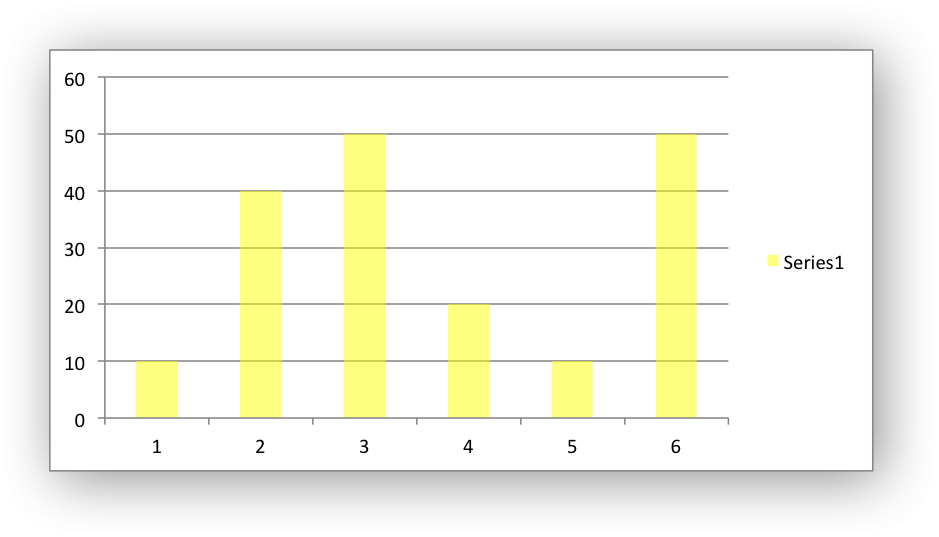The `fill` format is generally used in conjunction with a border which can be set via the `line` format: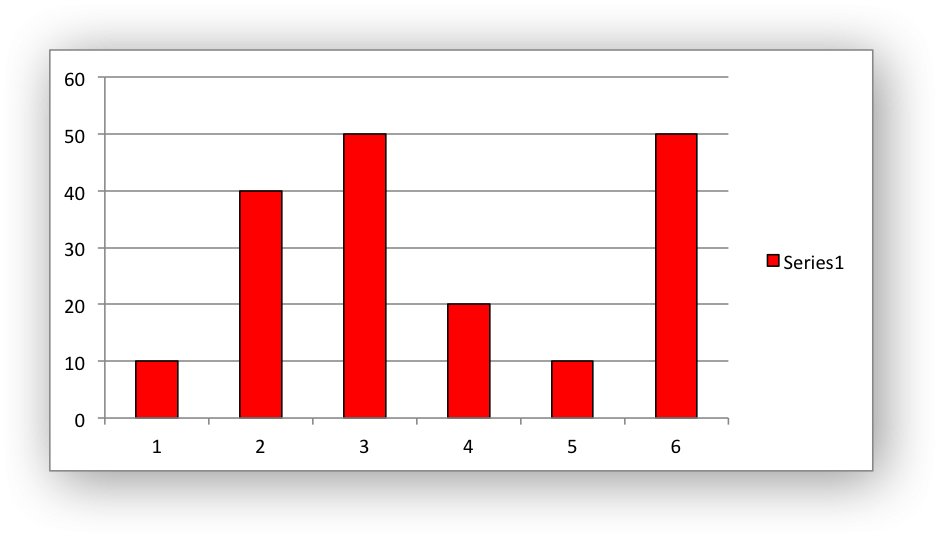## Chart formatting: Pattern

The pattern fill is used to specify pattern filled areas of chart objects such as the interior of a column or the background of the chart itself.

A chart pattern lxw_chart_pattern struct with default properties is:

.fg_color = LXW_COLOR_NONE,;
.bg_color = LXW_COLOR_WHITE};

Where the members are:

• `pattern`: The pattern to be applied (required).
• `fg_color`: The foreground color of the pattern (required).
• `bg_color`: The background color (optional, defaults to white).

The foreground color, `fg_color`, is a required parameter and can be HTML style RGB color or a limited number of named colors (see Working with Colors for more information):

The background color, `bg_color`, is optional and defaults to LXW_COLOR_WHITE.

For example:

.fg_color = 0x804000,
.bg_color = 0XC68C53};
.fg_color = 0XB30000,
.bg_color = 0XFF6666};
chart_series_set_pattern(series1, &pattern1);
chart_series_set_pattern(series2, &pattern2);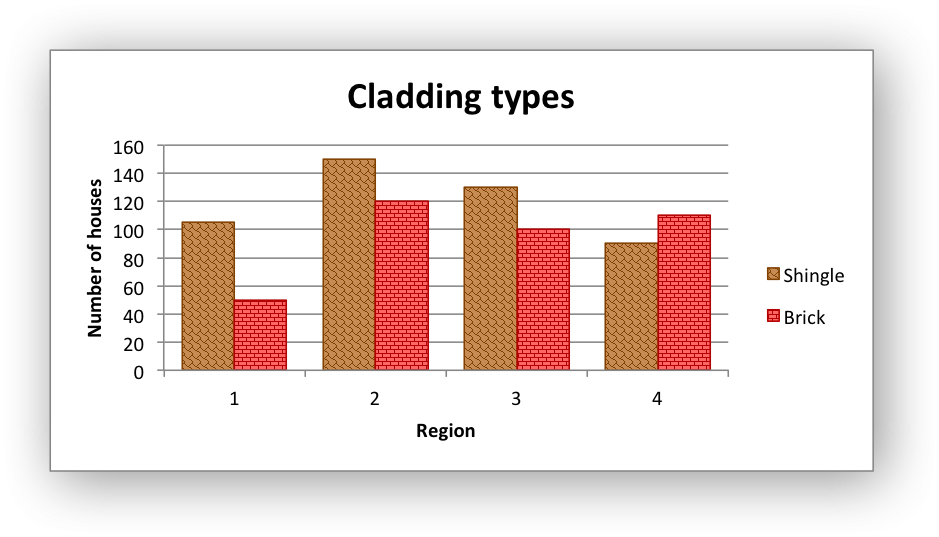The following patterns lxw_chart_pattern_type can be applied:

Note
If a pattern fill is used on a chart object it overrides the solid fill properties of the object.

# Chart Limitations

The following chart features aren't currently supported in libxlsxwriter but will be in time. See the GitHub Chart Feature Requests.

• Secondary axis.
• Combined charts.
• Chartsheets.

If required these features are all available in the Perl and Python versions of this library.

chart_series_set_marker_type
void chart_series_set_marker_type(lxw_chart_series *series, uint8_t type)
Set the data marker type for a series.
LXW_CHART_LINE_DASH_LONG_DASH
@ LXW_CHART_LINE_DASH_LONG_DASH
Definition: chart.h:218
lxw_chart_pattern
Struct to represent a chart pattern.
Definition: chart.h:681
chart_series_set_trendline_equation
void chart_series_set_trendline_equation(lxw_chart_series *series)
Display the equation of a trendline for a chart data series.
chart_series_set_marker_fill
void chart_series_set_marker_fill(lxw_chart_series *series, lxw_chart_fill *fill)
Set the fill properties for a chart series marker.
workbook_close
lxw_error workbook_close(lxw_workbook *workbook)
Close the Workbook object and write the XLSX file.
chart_series_set_trendline
void chart_series_set_trendline(lxw_chart_series *series, uint8_t type, uint8_t value)
Turn on a trendline for a chart data series.
chart_series_set_points
lxw_error chart_series_set_points(lxw_chart_series *series, lxw_chart_point *points[])
Set the formatting for points in the series.
chart_series_set_labels_custom
lxw_error chart_series_set_labels_custom(lxw_chart_series *series, lxw_chart_data_label *data_labels[])
Set the properties for data labels in a series.
chart_series_set_pattern
void chart_series_set_pattern(lxw_chart_series *series, lxw_chart_pattern *pattern)
Set the pattern properties for a chart series.
LXW_COLOR_WHITE
@ LXW_COLOR_WHITE
Definition: format.h:223
LXW_CHART_LABEL_SEPARATOR_NEWLINE
@ LXW_CHART_LABEL_SEPARATOR_NEWLINE
Definition: chart.h:473
chart_series_set_trendline_line
void chart_series_set_trendline_line(lxw_chart_series *series, lxw_chart_line *line)
Set the trendline line properties for a chart data series.
chart_series_set_trendline_forecast
void chart_series_set_trendline_forecast(lxw_chart_series *series, double forward, double backward)
Set the trendline forecast for a chart data series.
LXW_CHART_ERROR_BAR_NO_CAP
@ LXW_CHART_ERROR_BAR_NO_CAP
Definition: chart.h:914
chart_series_set_labels_legend
void chart_series_set_labels_legend(lxw_chart_series *series)
Set the legend key for a data label in a chart series.
LXW_CHART_ERROR_BAR_TYPE_STD_DEV
@ LXW_CHART_ERROR_BAR_TYPE_STD_DEV
Definition: chart.h:877
LXW_CHART_MARKER_CIRCLE
@ LXW_CHART_MARKER_CIRCLE
Definition: chart.h:265
lxw_chart_line::width
float width
Definition: chart.h:648
chart_series_set_labels_font
void chart_series_set_labels_font(lxw_chart_series *series, lxw_chart_font *font)
Set the font properties for chart data labels in a series.
chart_legend_set_font
void chart_legend_set_font(lxw_chart *chart, lxw_chart_font *font)
Set the font properties for a chart legend.
LXW_FALSE
@ LXW_FALSE
Definition: common.h:51
LXW_CHART_PIE
@ LXW_CHART_PIE
Definition: chart.h:134
chart_axis_set_name
void chart_axis_set_name(lxw_chart_axis *axis, const char *name)
Set the name caption of the an axis.
LXW_CHART_TRENDLINE_TYPE_AVERAGE
@ LXW_CHART_TRENDLINE_TYPE_AVERAGE
Definition: chart.h:950
lxw_chart_data_label::value
char * value
Definition: chart.h:803
lxw_chart_series * chart_add_series(lxw_chart *chart, const char *categories, const char *values)
Add a data series to a chart.
LXW_CHART_PATTERN_HORIZONTAL_BRICK
@ LXW_CHART_PATTERN_HORIZONTAL_BRICK
Definition: chart.h:379
workbook_new
lxw_workbook * workbook_new(const char *filename)
Create a new workbook object.
LXW_CHART_LINE_DASH_SQUARE_DOT
@ LXW_CHART_LINE_DASH_SQUARE_DOT
Definition: chart.h:209
lxw_chart_font::color
lxw_color_t color
Definition: chart.h:726
LXW_CHART_ERROR_BAR_TYPE_STD_ERROR
@ LXW_CHART_ERROR_BAR_TYPE_STD_ERROR
Definition: chart.h:868
chart_series_set_labels_percentage
void chart_series_set_labels_percentage(lxw_chart_series *series)
Set the percentage for a Pie/Doughnut data point.
chart_title_set_name_font
void chart_title_set_name_font(lxw_chart *chart, lxw_chart_font *font)
Set the font properties for a chart title.
chart_series_set_error_bars
void chart_series_set_error_bars(lxw_series_error_bars *error_bars, uint8_t type, double value)
LXW_CHART_ERROR_BAR_TYPE_PERCENTAGE
@ LXW_CHART_ERROR_BAR_TYPE_PERCENTAGE
Definition: chart.h:874
lxw_chart_font::italic
uint8_t italic
Definition: chart.h:711
lxw_chart_line
Struct to represent a chart line.
Definition: chart.h:639
chart_series_set_trendline_intercept
void chart_series_set_trendline_intercept(lxw_chart_series *series, double intercept)
Set the trendline Y-axis intercept for a chart data series.
chart_series_set_labels_line
void chart_series_set_labels_line(lxw_chart_series *series, lxw_chart_line *line)
Set the line properties for the data labels in a chart series.
LXW_COLOR_YELLOW
@ LXW_COLOR_YELLOW
Definition: format.h:226
lxw_chart_font
Struct to represent a chart font.
Definition: chart.h:699
LXW_CHART_LINE
@ LXW_CHART_LINE
Definition: chart.h:125
lxw_worksheet
Struct to represent an Excel worksheet.
Definition: worksheet.h:2107
chart_series_set_marker_line
void chart_series_set_marker_line(lxw_chart_series *series, lxw_chart_line *line)
Set the line properties for a chart series marker.
LXW_COLOR_BLACK
@ LXW_COLOR_BLACK
Definition: format.h:181
lxw_chart_data_label
Struct to represent an Excel chart data label.
Definition: chart.h:799
chart_series_set_error_bars_line
void chart_series_set_error_bars_line(lxw_series_error_bars *error_bars, lxw_chart_line *line)
Set the line properties for a chart series error bars.
chart_series_set_trendline_name
void chart_series_set_trendline_name(lxw_chart_series *series, const char *name)
Set the trendline name for a chart data series.
chart_axis_set_name_font
void chart_axis_set_name_font(lxw_chart_axis *axis, lxw_chart_font *font)
Set the font properties for a chart axis name.
LXW_CHART_LINE_DASH_DASH_DOT
@ LXW_CHART_LINE_DASH_DASH_DOT
Definition: chart.h:215
lxw_chart_point::fill
lxw_chart_fill * fill
Definition: chart.h:786
LXW_TRUE
@ LXW_TRUE
Definition: common.h:53
LXW_CHART_TRENDLINE_TYPE_LINEAR
@ LXW_CHART_TRENDLINE_TYPE_LINEAR
Definition: chart.h:935
lxw_chart
Struct to represent an Excel chart.
Definition: chart.h:1090
worksheet_insert_chart
lxw_error worksheet_insert_chart(lxw_worksheet *worksheet, lxw_row_t row, lxw_col_t col, lxw_chart *chart)
Insert a chart object into a worksheet.
lxw_chart_series
Struct to represent an Excel chart data series.
Definition: chart.h:960
lxw_chart_line::dash_type
uint8_t dash_type
Definition: chart.h:651
lxw_chart_fill::color
lxw_color_t color
Definition: chart.h:666
LXW_CHART_LABEL_POSITION_ABOVE
@ LXW_CHART_LABEL_POSITION_ABOVE
Definition: chart.h:441
chart_series_set_error_bars_endcap
void chart_series_set_error_bars_endcap(lxw_series_error_bars *error_bars, uint8_t endcap)
Set the end cap type for the error bars of a chart series.
chart_series_set_marker_size
void chart_series_set_marker_size(lxw_chart_series *series, uint8_t size)
Set the size of a data marker for a series.
lxw_chart_line::none
uint8_t none
Definition: chart.h:645
lxw_chart::y_axis
lxw_chart_axis * y_axis
Definition: chart.h:1111
LXW_CHART_MARKER_DIAMOND
@ LXW_CHART_MARKER_DIAMOND
Definition: chart.h:247
chart_series_set_error_bars_direction
void chart_series_set_error_bars_direction(lxw_series_error_bars *error_bars, uint8_t direction)
Set the direction (up, down or both) of the error bars for a chart series.
chart_series_set_labels_position
void chart_series_set_labels_position(lxw_chart_series *series, uint8_t position)
Set the data label position for a series.
chart_legend_delete_series
lxw_error chart_legend_delete_series(lxw_chart *chart, int16_t delete_series[])
Remove one or more series from the the legend.
LXW_COLOR_GREEN
@ LXW_COLOR_GREEN
Definition: format.h:196
chart_series_set_labels
void chart_series_set_labels(lxw_chart_series *series)
Add data labels to a chart series.
lxw_chart_fill
Struct to represent a chart fill.
Definition: chart.h:663
chart_series_set_trendline_r_squared
void chart_series_set_trendline_r_squared(lxw_chart_series *series)
Display the R squared value of a trendline for a chart data series.
LXW_CHART_LINE_DASH_SOLID
@ LXW_CHART_LINE_DASH_SOLID
Definition: chart.h:203
LXW_CHART_MARKER_AUTOMATIC
@ LXW_CHART_MARKER_AUTOMATIC
Definition: chart.h:238
chart_series_set_labels_separator
void chart_series_set_labels_separator(lxw_chart_series *series, uint8_t separator)
Set the separator for the data label captions.
lxw_chart_font::name
char * name
Definition: chart.h:702
lxw_chart_line::color
lxw_color_t color
Definition: chart.h:642
lxw_workbook
Struct to represent an Excel workbook.
Definition: workbook.h:292
lxw_chart::x_axis
lxw_chart_axis * x_axis
Definition: chart.h:1105
chart_series_set_fill
void chart_series_set_fill(lxw_chart_series *series, lxw_chart_fill *fill)
Set the fill properties for a chart series.
lxw_chart_font::rotation
int32_t rotation
Definition: chart.h:723
lxw_chart_fill::none
uint8_t none
Definition: chart.h:669
chart_legend_set_position
void chart_legend_set_position(lxw_chart *chart, uint8_t position)
Set the position of the chart legend.
LXW_CHART_ERROR_BAR_TYPE_FIXED
@ LXW_CHART_ERROR_BAR_TYPE_FIXED
Definition: chart.h:871
LXW_CHART_PATTERN_SHINGLE
@ LXW_CHART_PATTERN_SHINGLE
Definition: chart.h:397
chart_series_set_labels_options
void chart_series_set_labels_options(lxw_chart_series *series, uint8_t show_name, uint8_t show_category, uint8_t show_value)
Set the display options for the labels of a data series.
chart_title_set_name
void chart_title_set_name(lxw_chart *chart, const char *name)
Set the title of the chart.
chart_series_set_line
void chart_series_set_line(lxw_chart_series *series, lxw_chart_line *line)
Set the line properties for a chart series.
LXW_COLOR_BLUE
@ LXW_COLOR_BLUE
Definition: format.h:184
lxw_chart_font::bold
uint8_t bold
Definition: chart.h:708
worksheet_write_string
lxw_error worksheet_write_string(lxw_worksheet *worksheet, lxw_row_t row, lxw_col_t col, const char *string, lxw_format *format)
Write a string to a worksheet cell.
LXW_CHART_PATTERN_NONE
@ LXW_CHART_PATTERN_NONE
Definition: chart.h:277
LXW_COLOR_RED
@ LXW_COLOR_RED
Definition: format.h:217
worksheet_write_number
lxw_error worksheet_write_number(lxw_worksheet *worksheet, lxw_row_t row, lxw_col_t col, double number, lxw_format *format)
Write a number to a worksheet cell.
LXW_CHART_MARKER_NONE
@ LXW_CHART_MARKER_NONE
Definition: chart.h:241
lxw_chart_data_label::hide
uint8_t hide
Definition: chart.h:807
LXW_CHART_LINE_DASH_ROUND_DOT
@ LXW_CHART_LINE_DASH_ROUND_DOT
Definition: chart.h:206
LXW_CHART_ERROR_BAR_DIR_PLUS
@ LXW_CHART_ERROR_BAR_DIR_PLUS
Definition: chart.h:889
lxw_chart_font::underline
uint8_t underline
Definition: chart.h:714
lxw_chart_pattern::type
uint8_t type
Definition: chart.h:690
LXW_CHART_LEGEND_BOTTOM
@ LXW_CHART_LEGEND_BOTTOM
Definition: chart.h:179
chart_series_set_labels_num_format
void chart_series_set_labels_num_format(lxw_chart_series *series, const char *num_format)
Set the number format for chart data labels in a series.
lxw_chart_point
Struct to represent an Excel chart data point.
Definition: chart.h:780
Set leader lines for Pie and Doughnut charts.
CELL
#define CELL(cell)
Convert an Excel A1 cell string into a (row, col) pair.
Definition: utility.h:45
LXW_CHART_MARKER_SQUARE
@ LXW_CHART_MARKER_SQUARE
Definition: chart.h:244